# Frank Solutions for Class 9 Maths Chapter 10 Logarithms

Frank Solutions for Class 9 Maths Chapter 10 Logarithms help students to understand the concepts covered in this chapter easily. The solutions are created by subject experts at BYJU’S with the aim of clearing students’ doubts instantly, at their convenience. Students who refer to these solutions during exam preparation will improve their problem-solving skills.

Chapter 10 mainly deals with the study of Logarithms. Students can cross-check their answers by referring to these solutions while solving exercise problems. Regular practice of these solutions will build confidence in students who find difficulty in solving textbook problems. In order to prepare well for the annual exams, students can download Frank Solutions for Class 9 Maths Chapter 10 Logarithms PDF from the link given below and practise regularly.

## Frank Solutions for Class 9 Maths Chapter 10 Logarithms Download Free PDF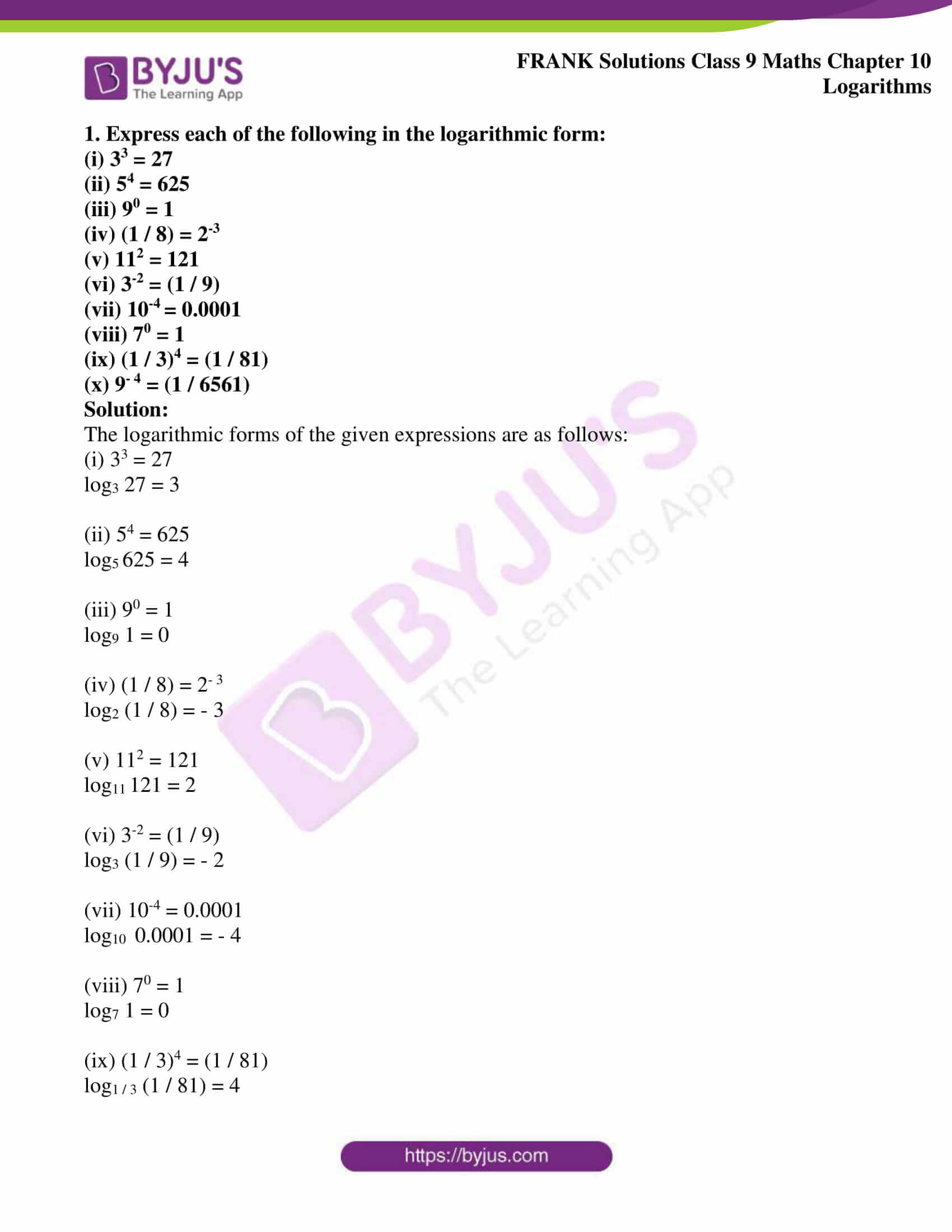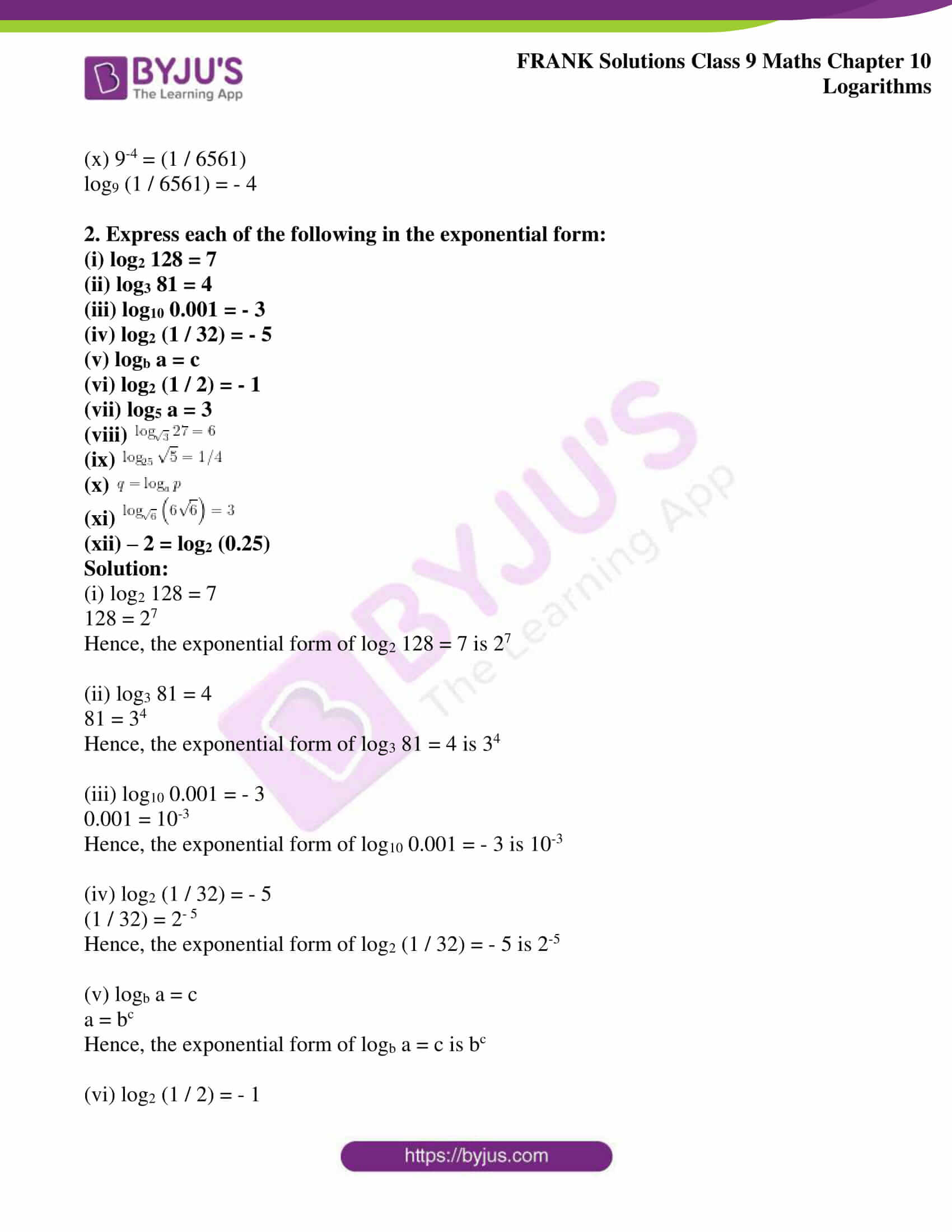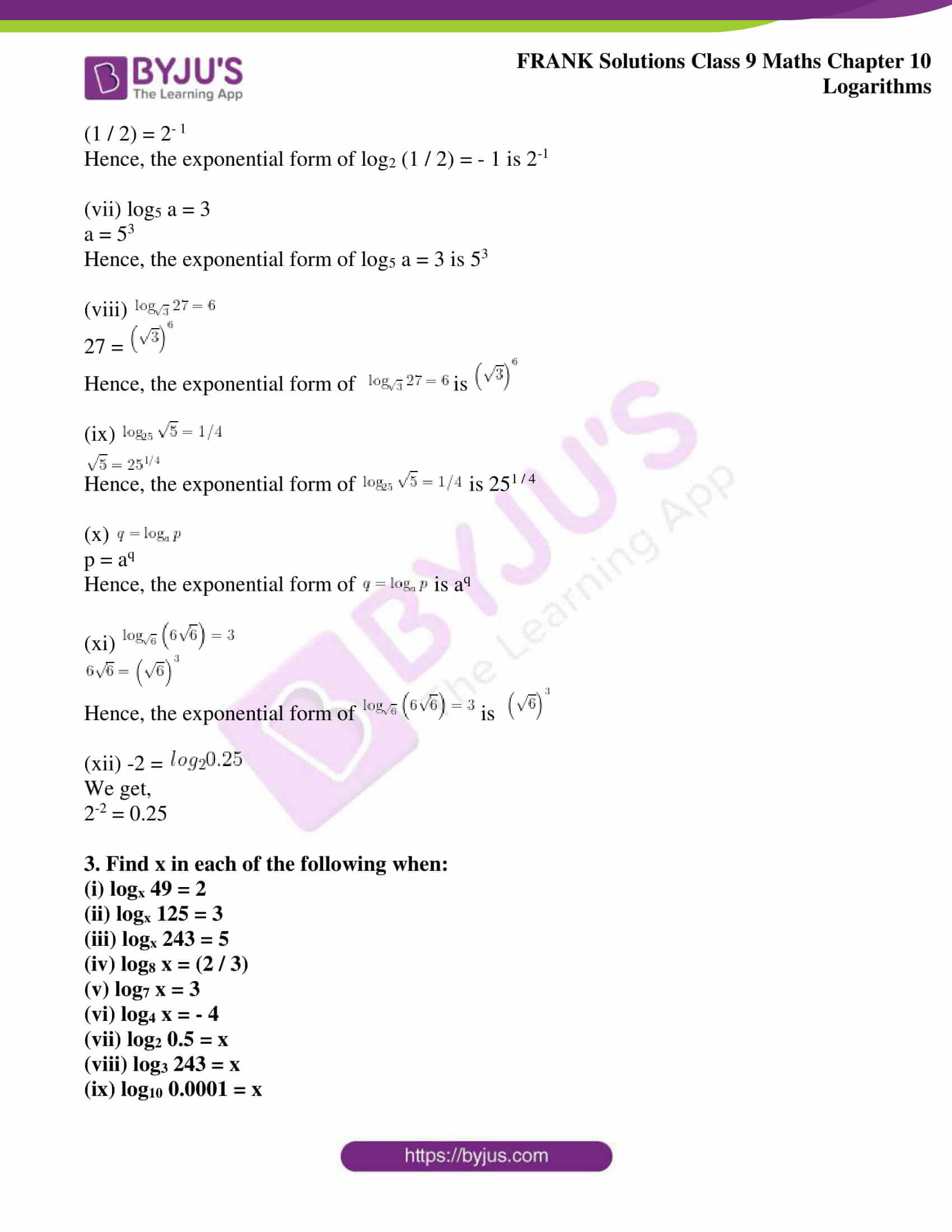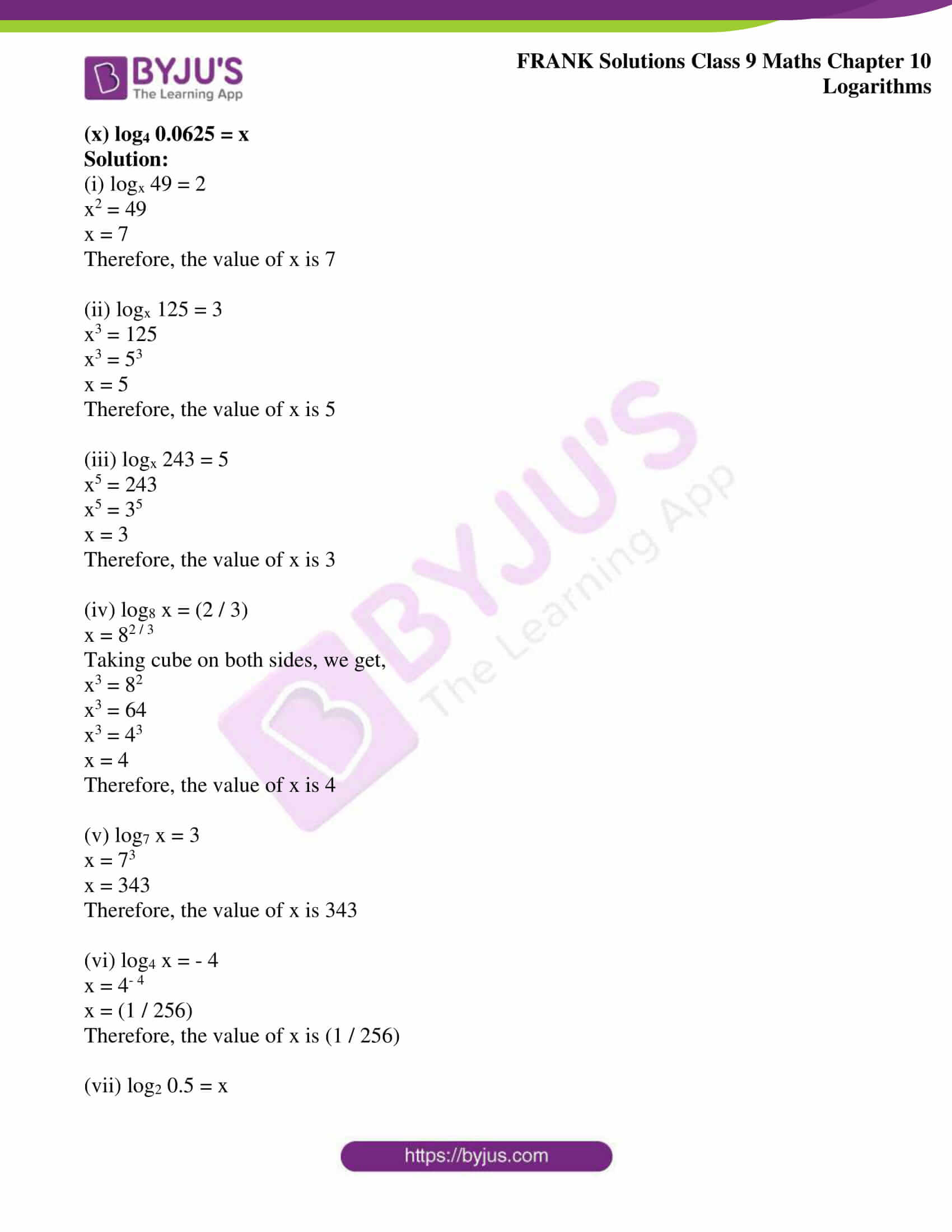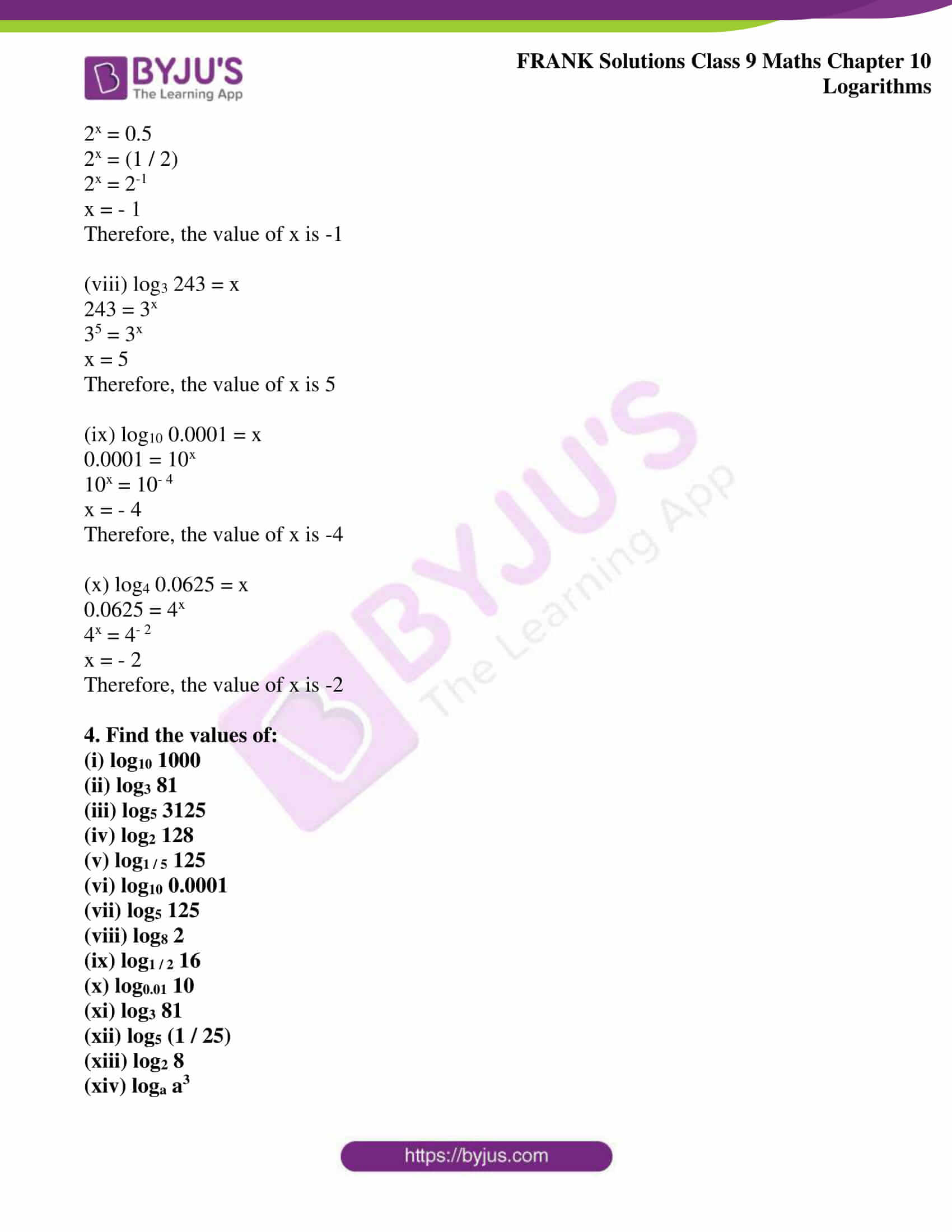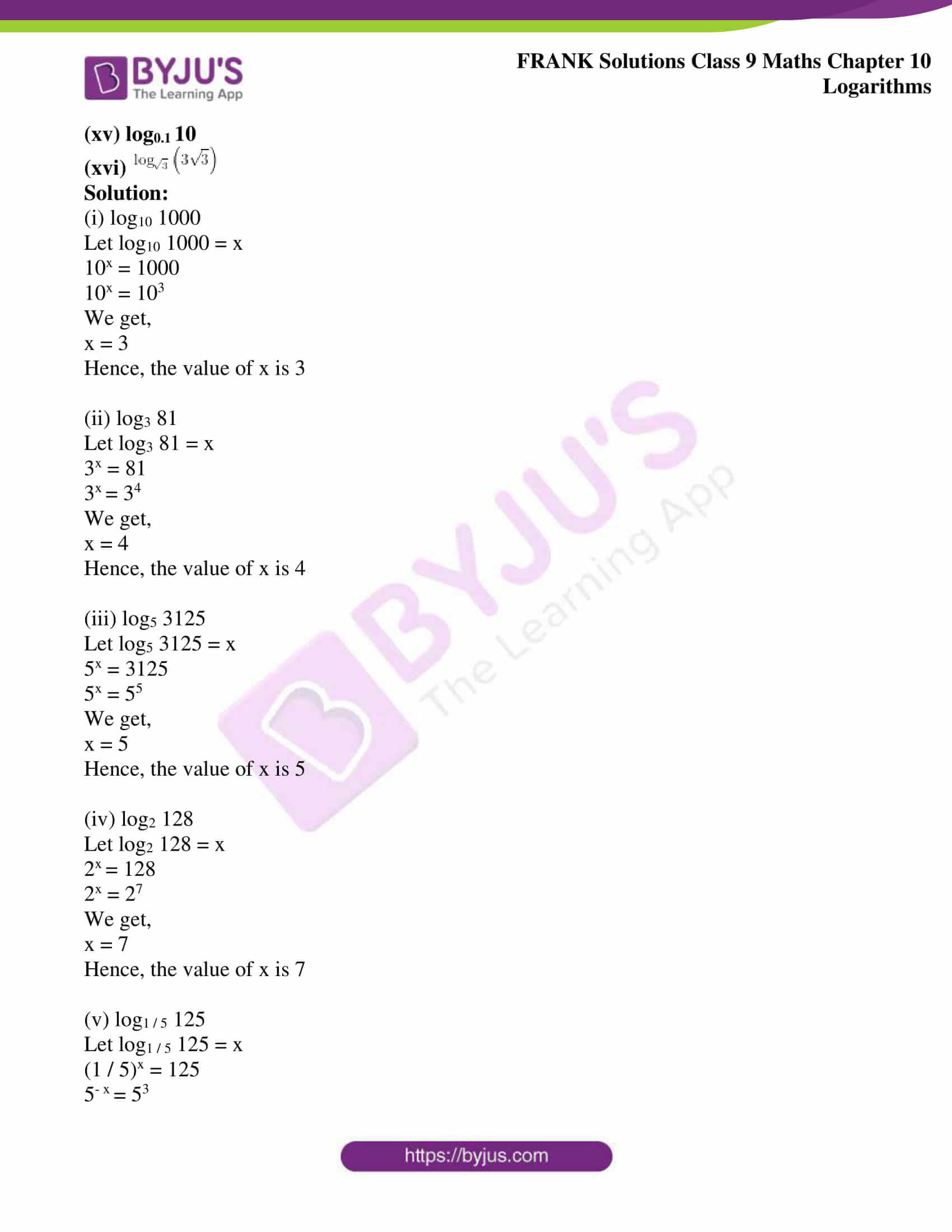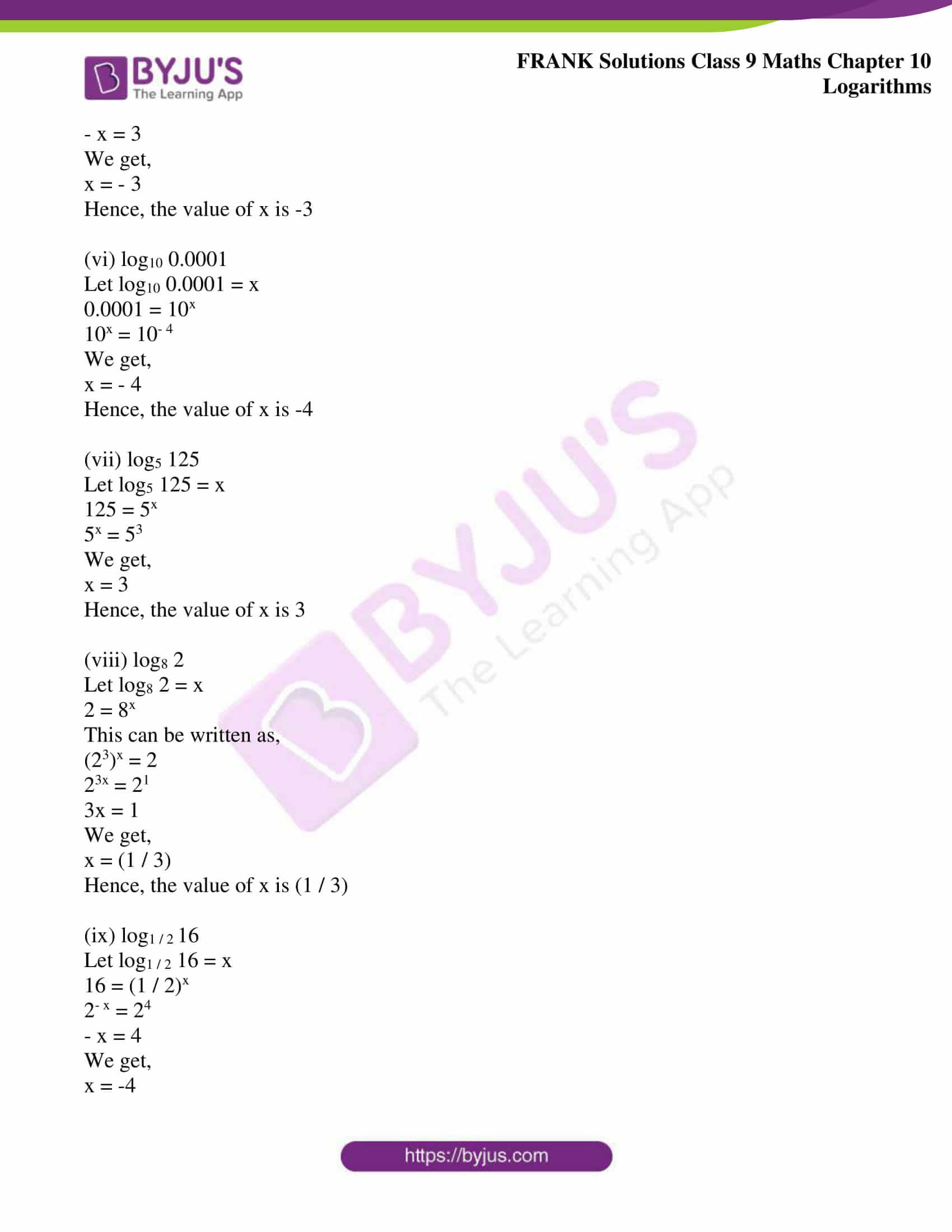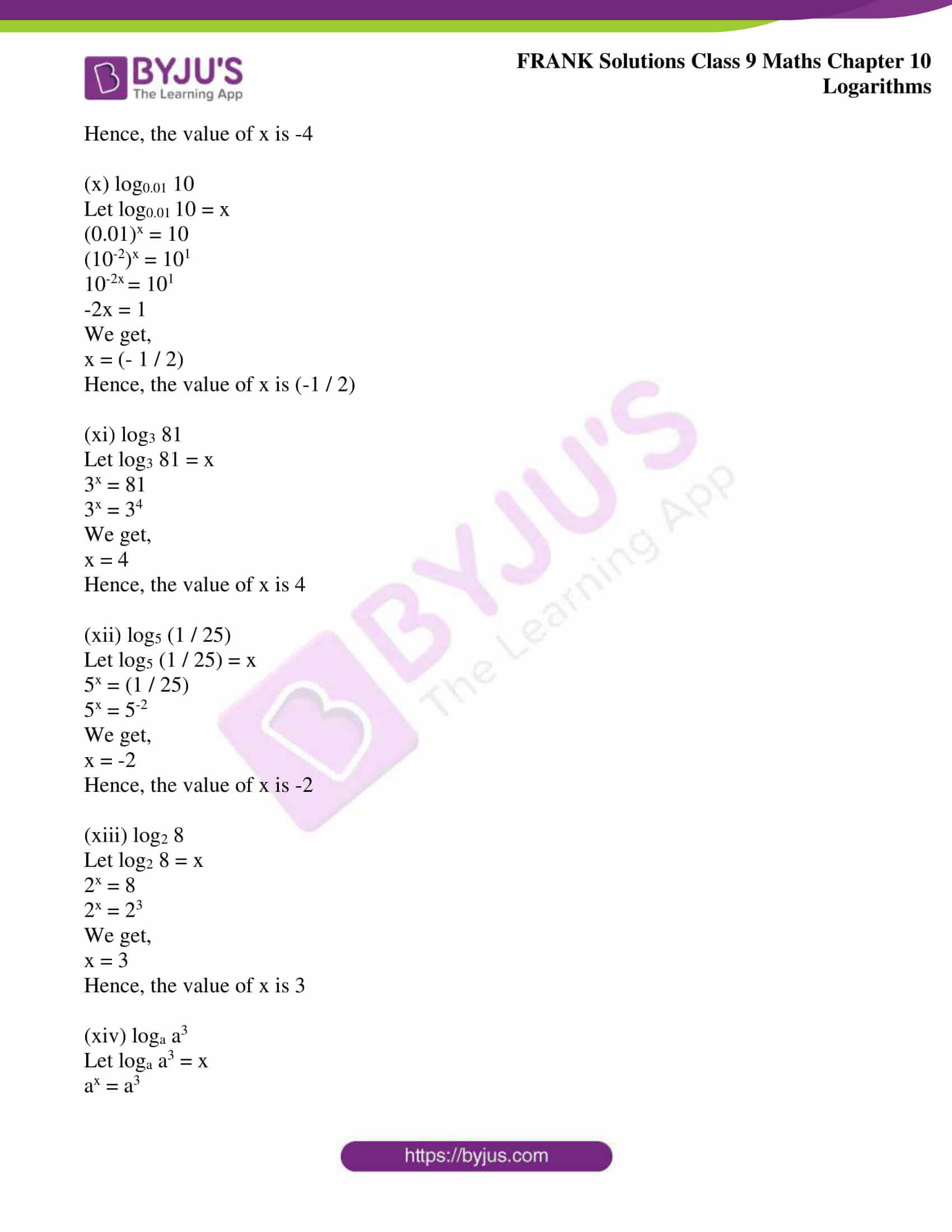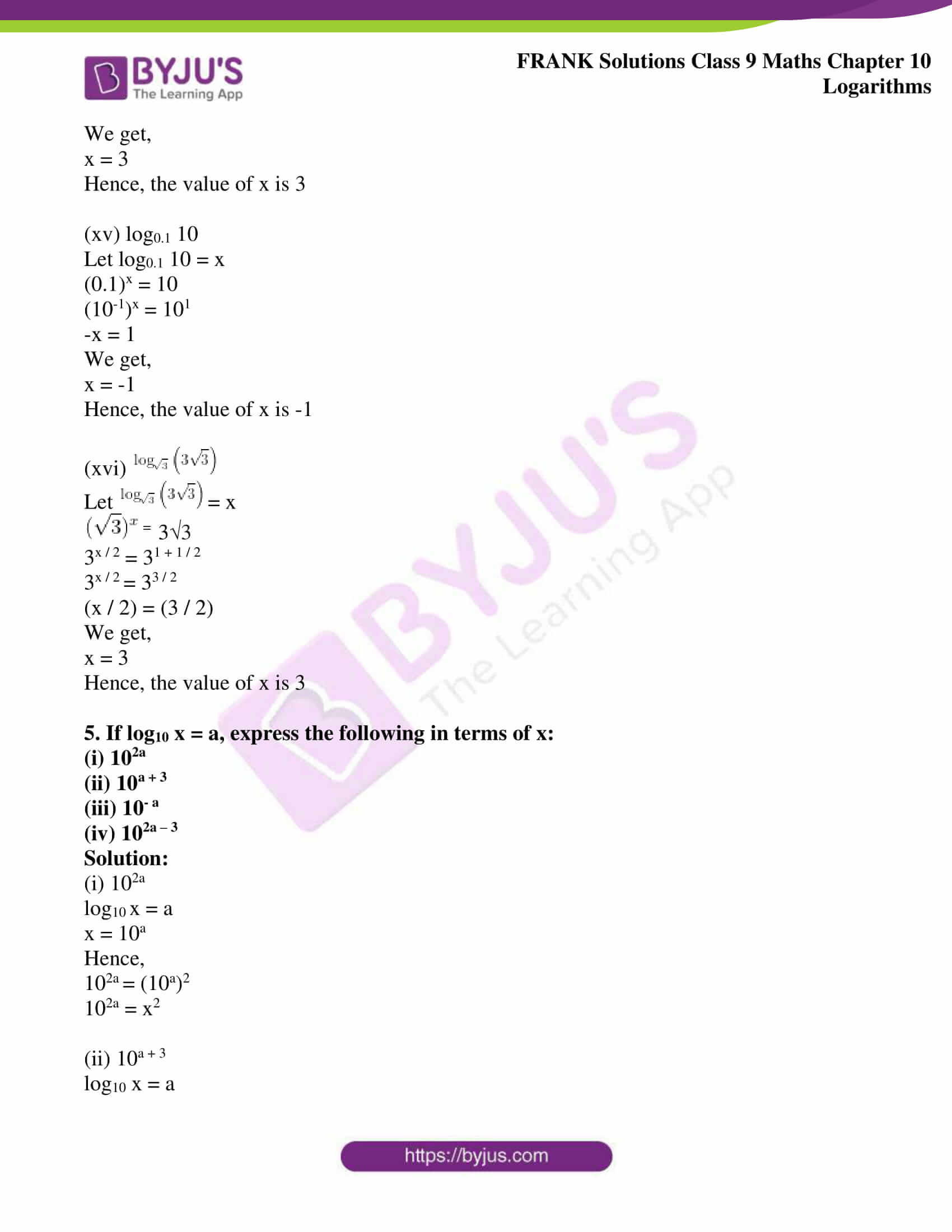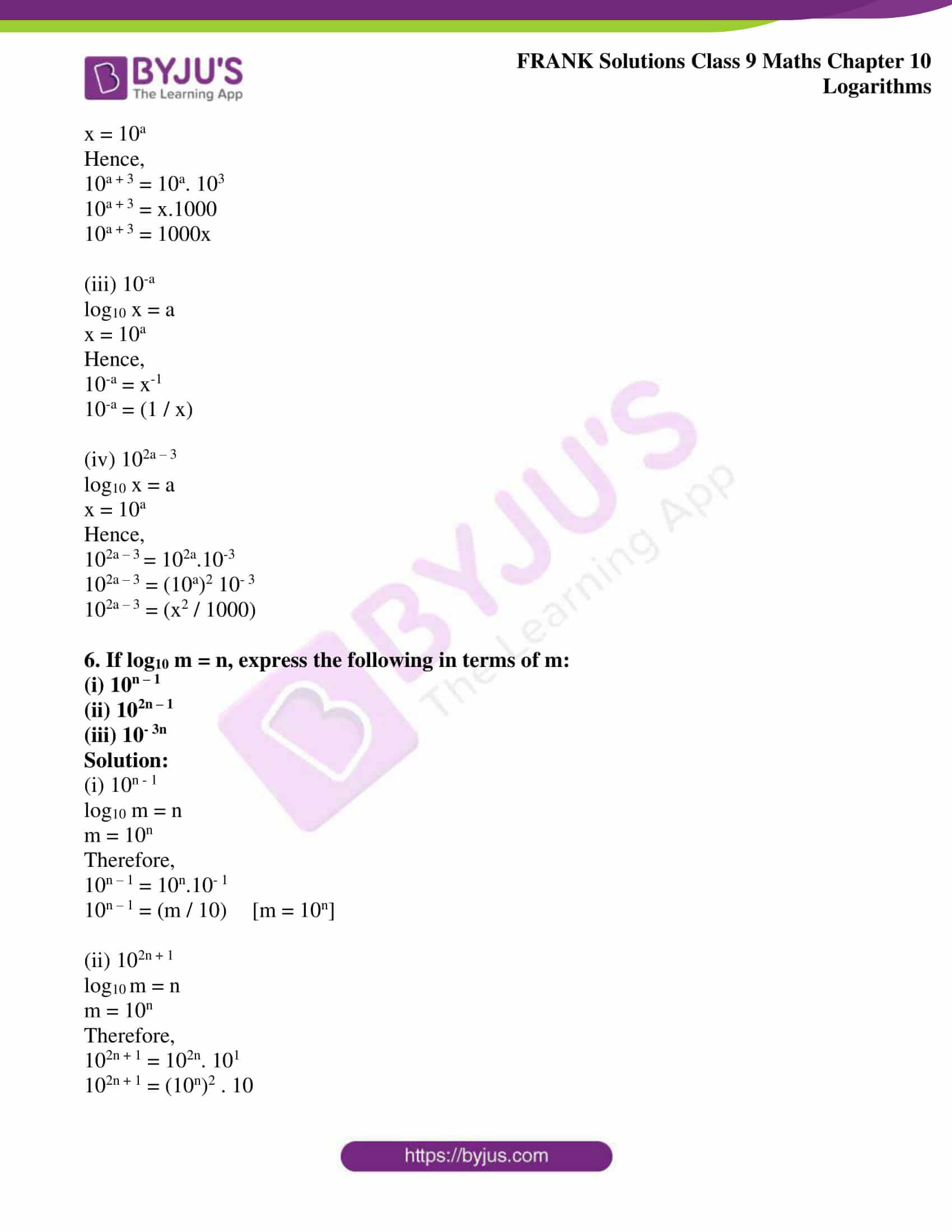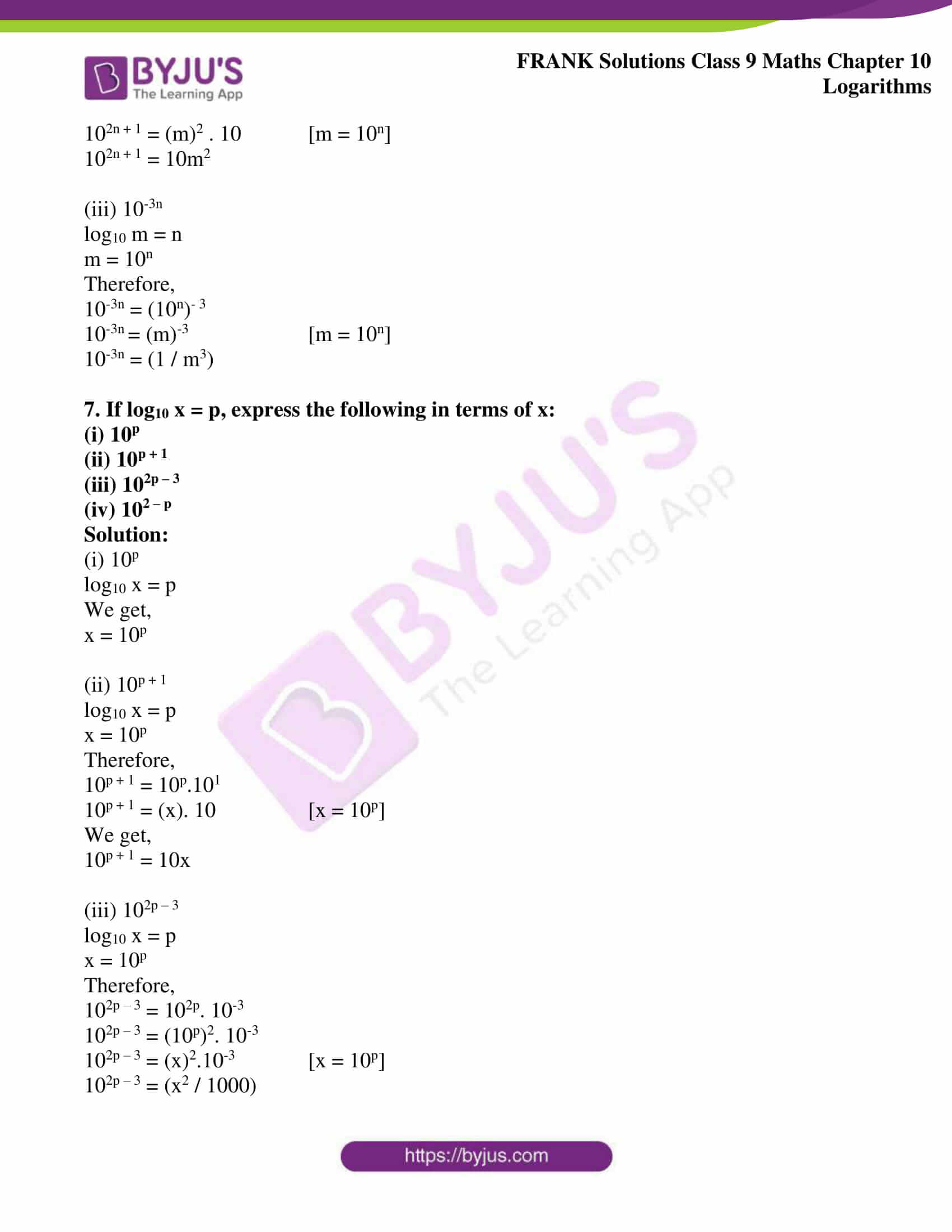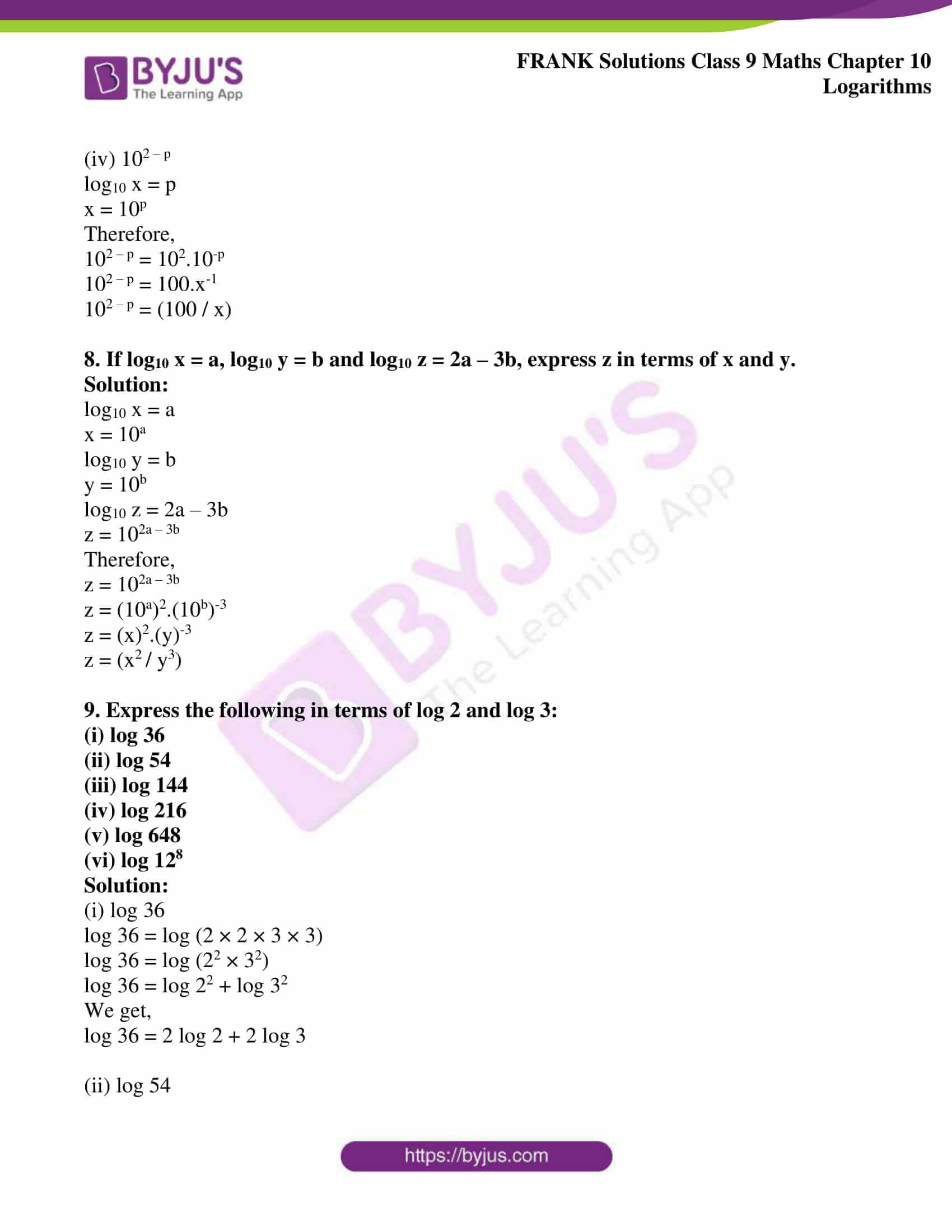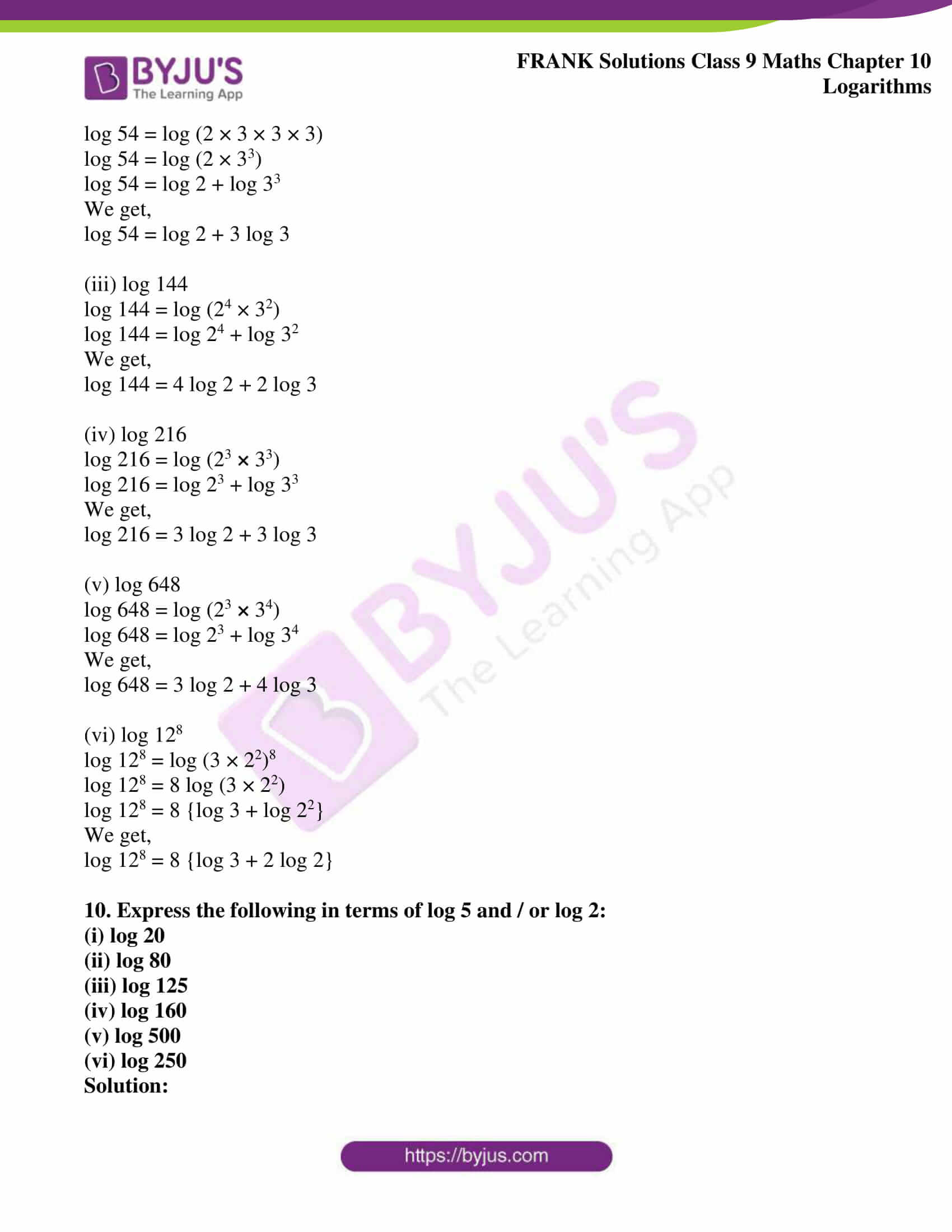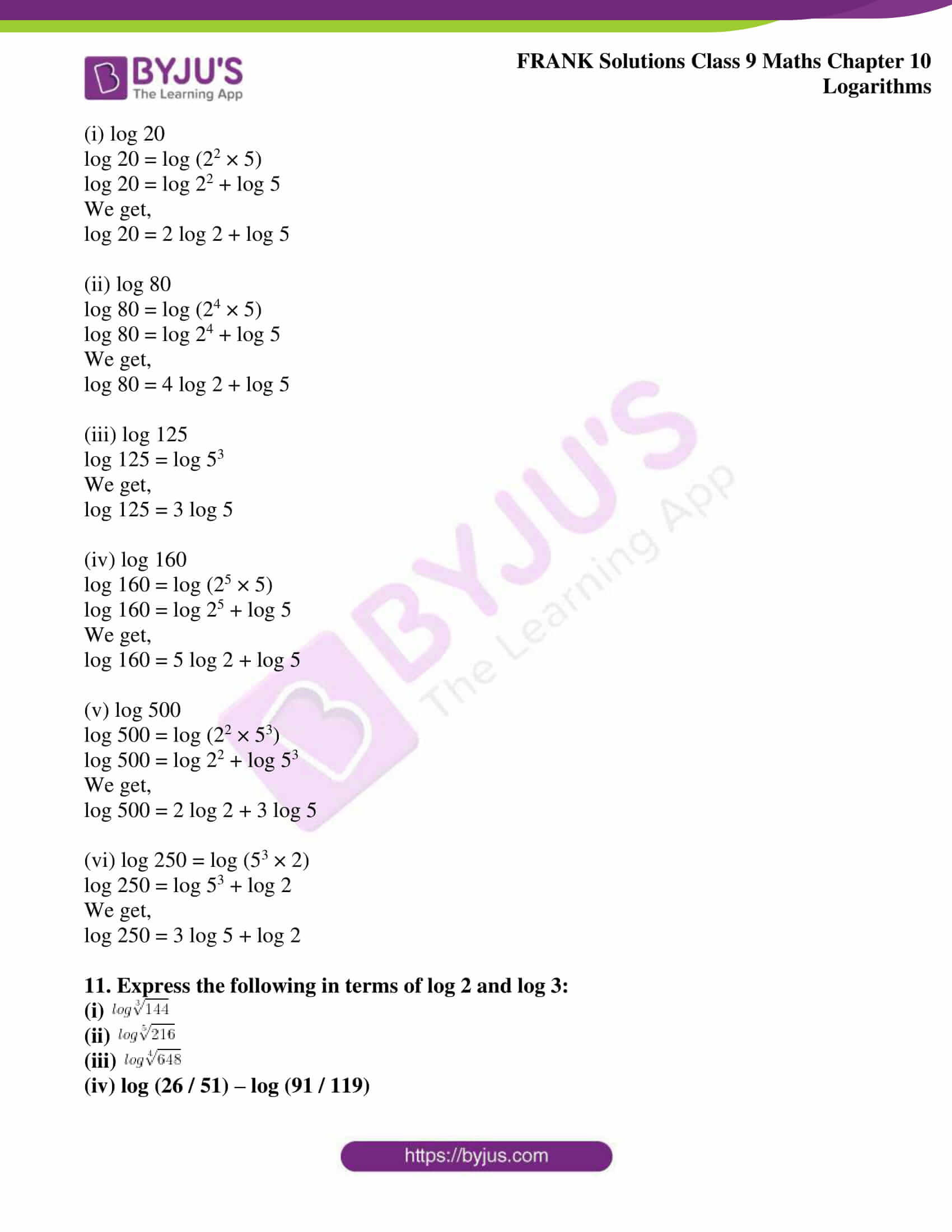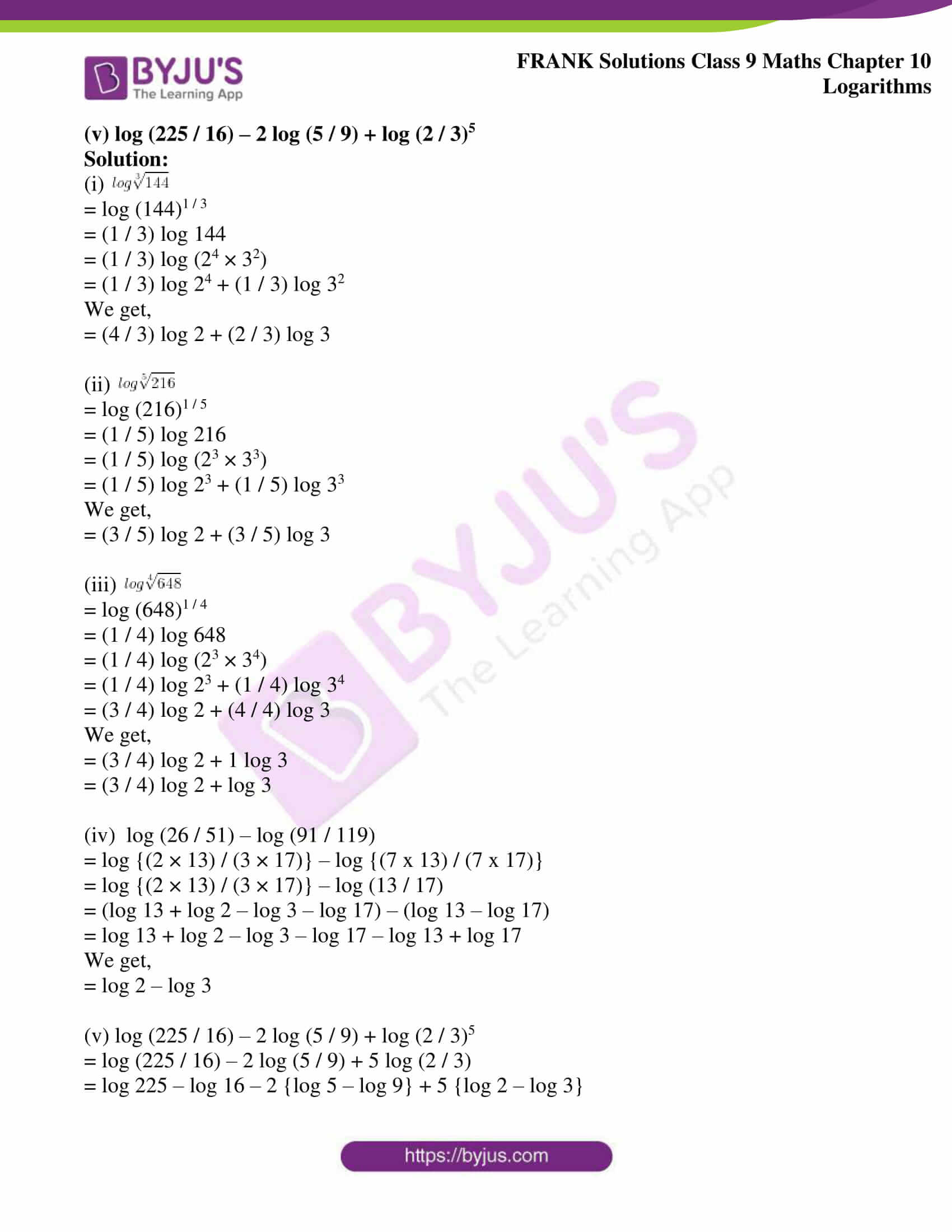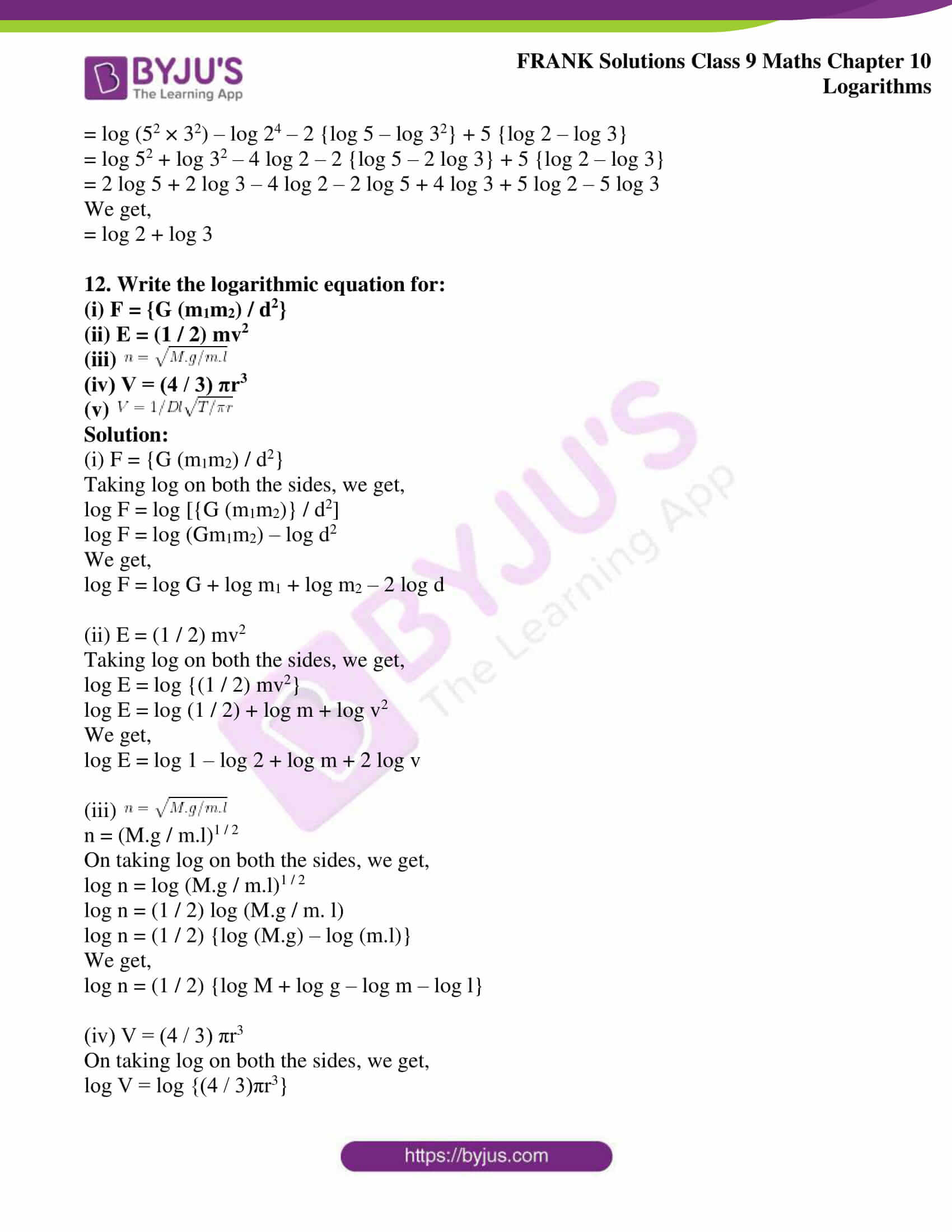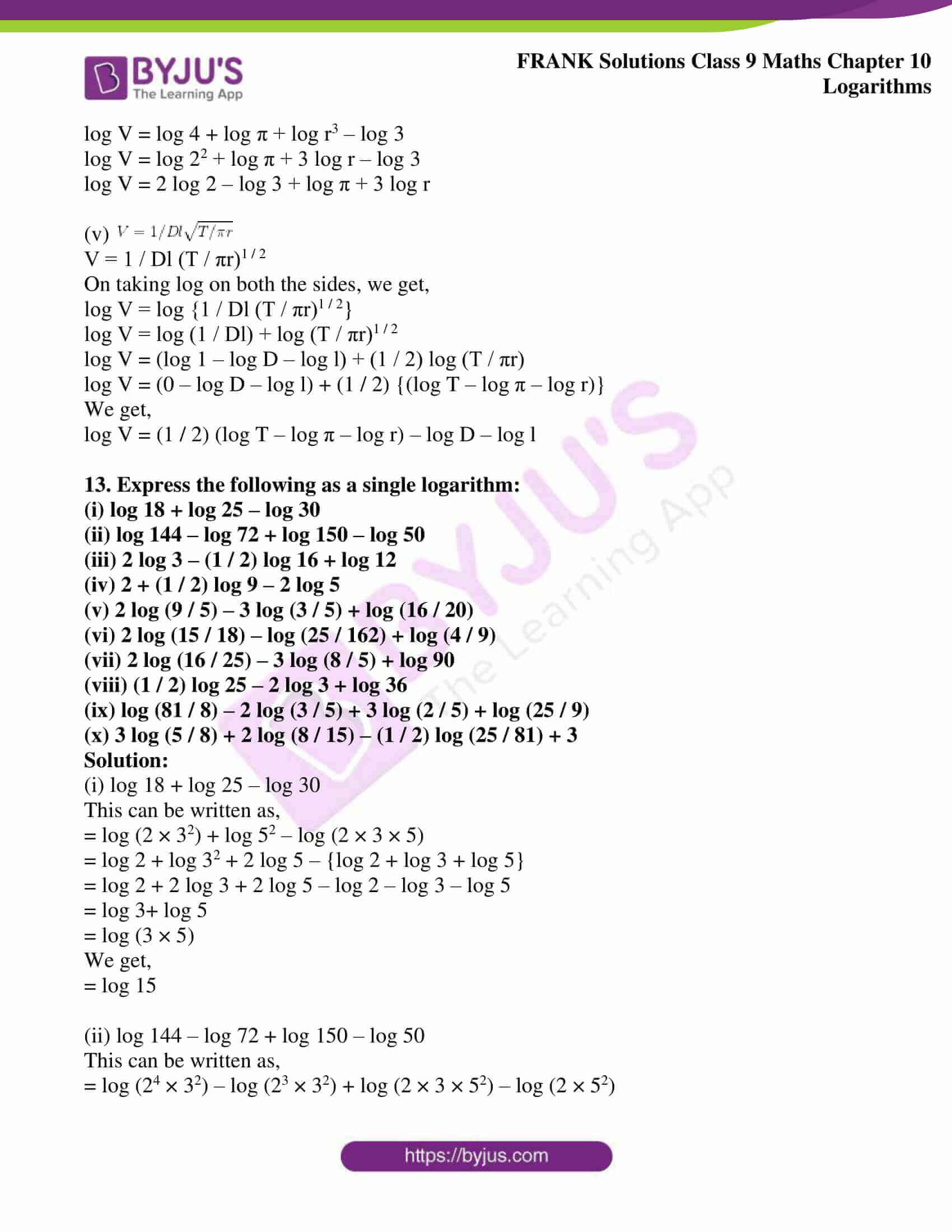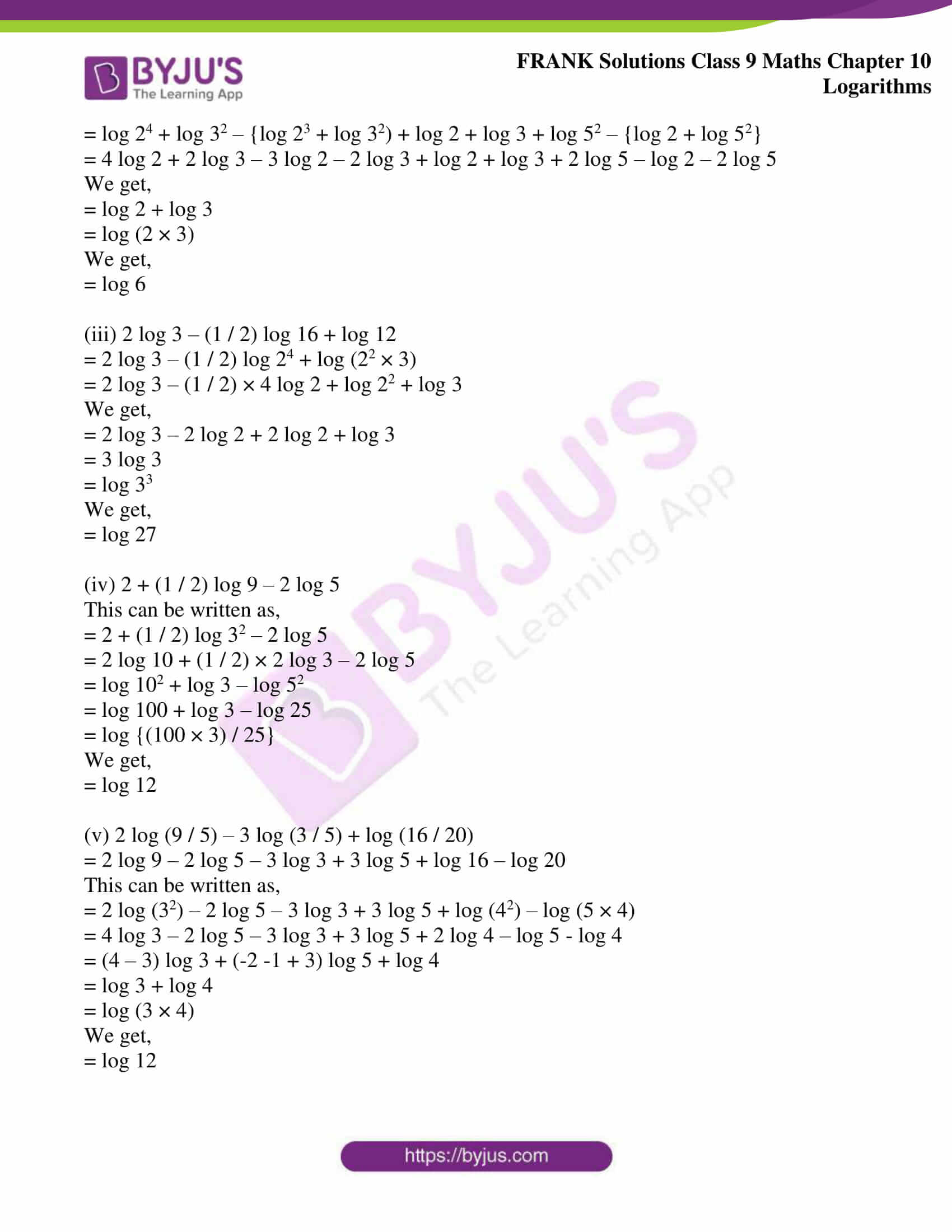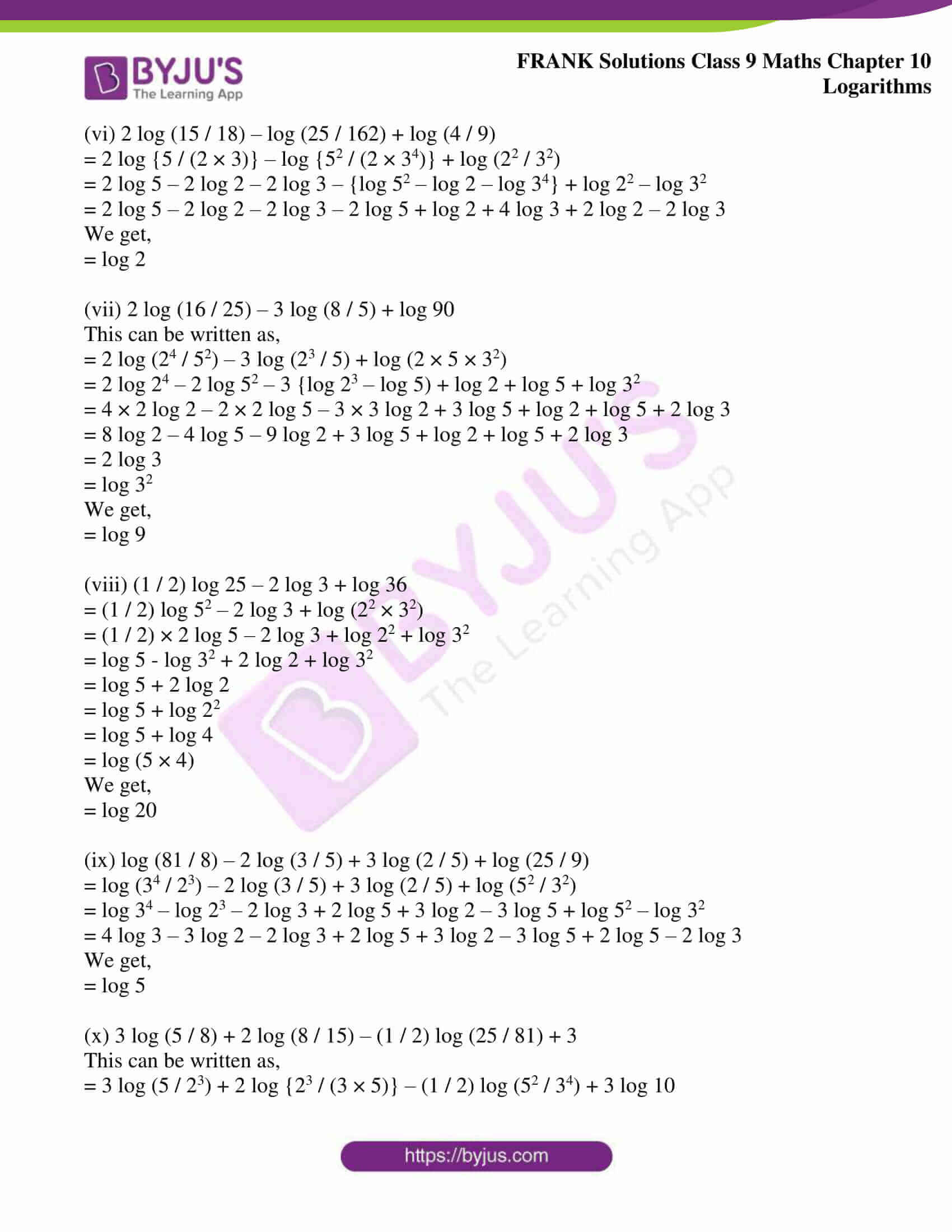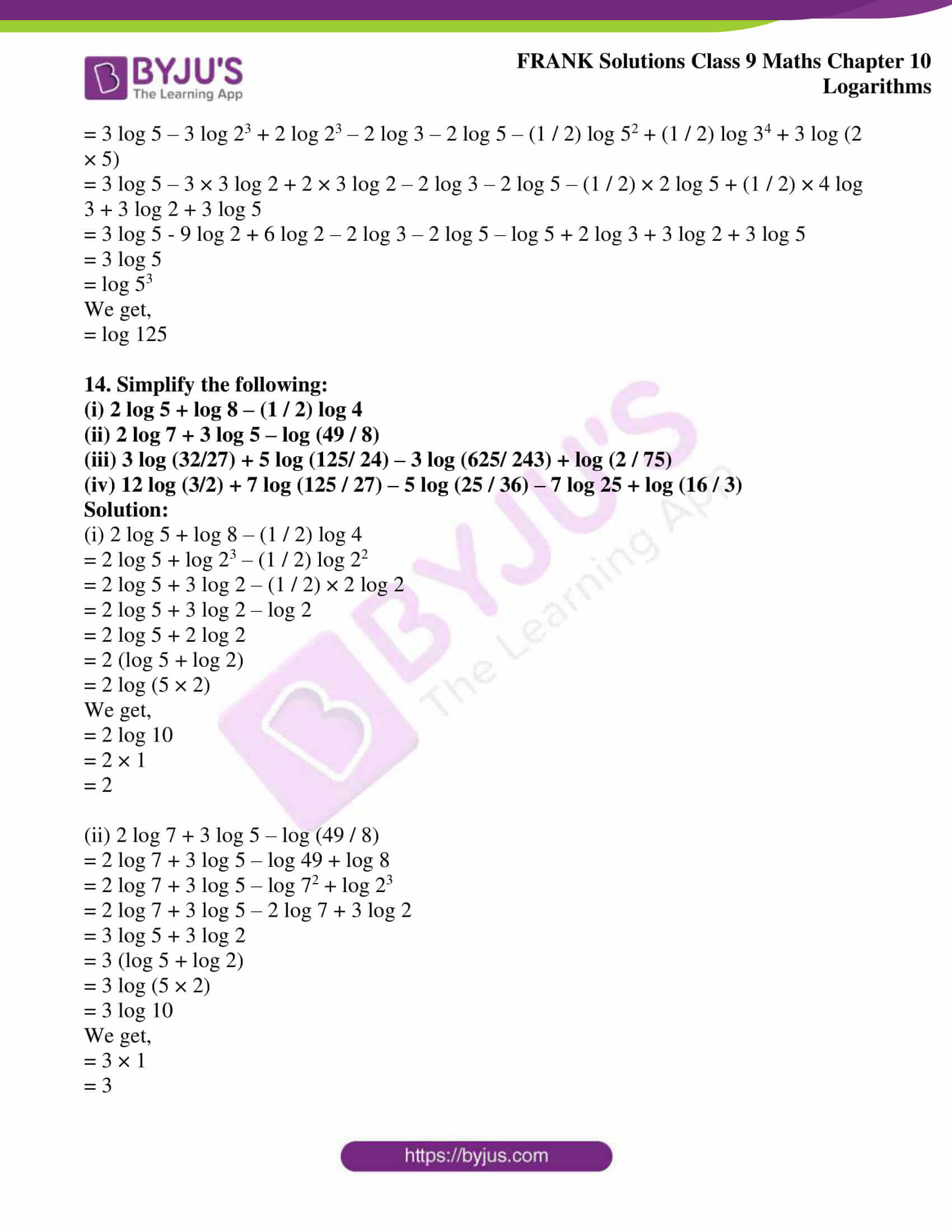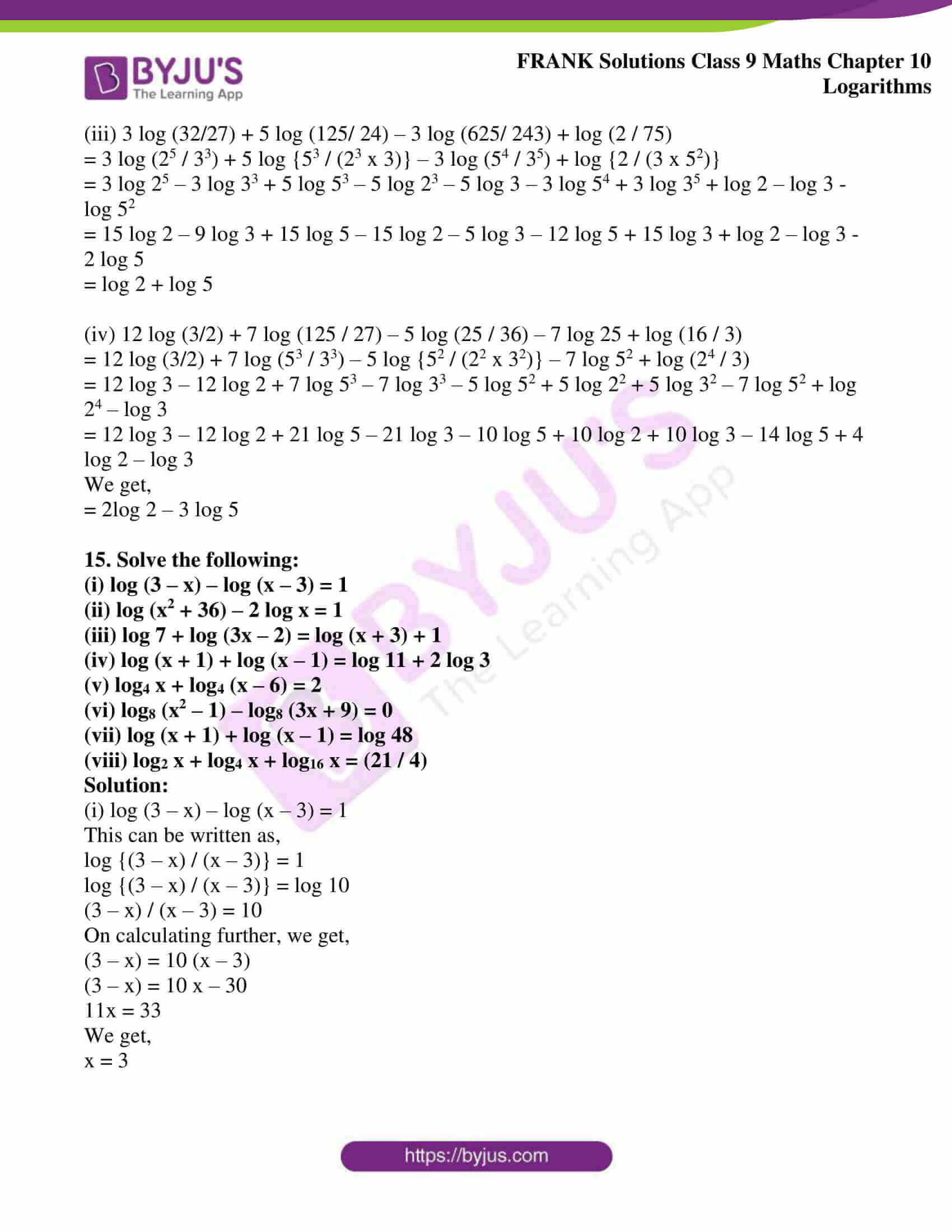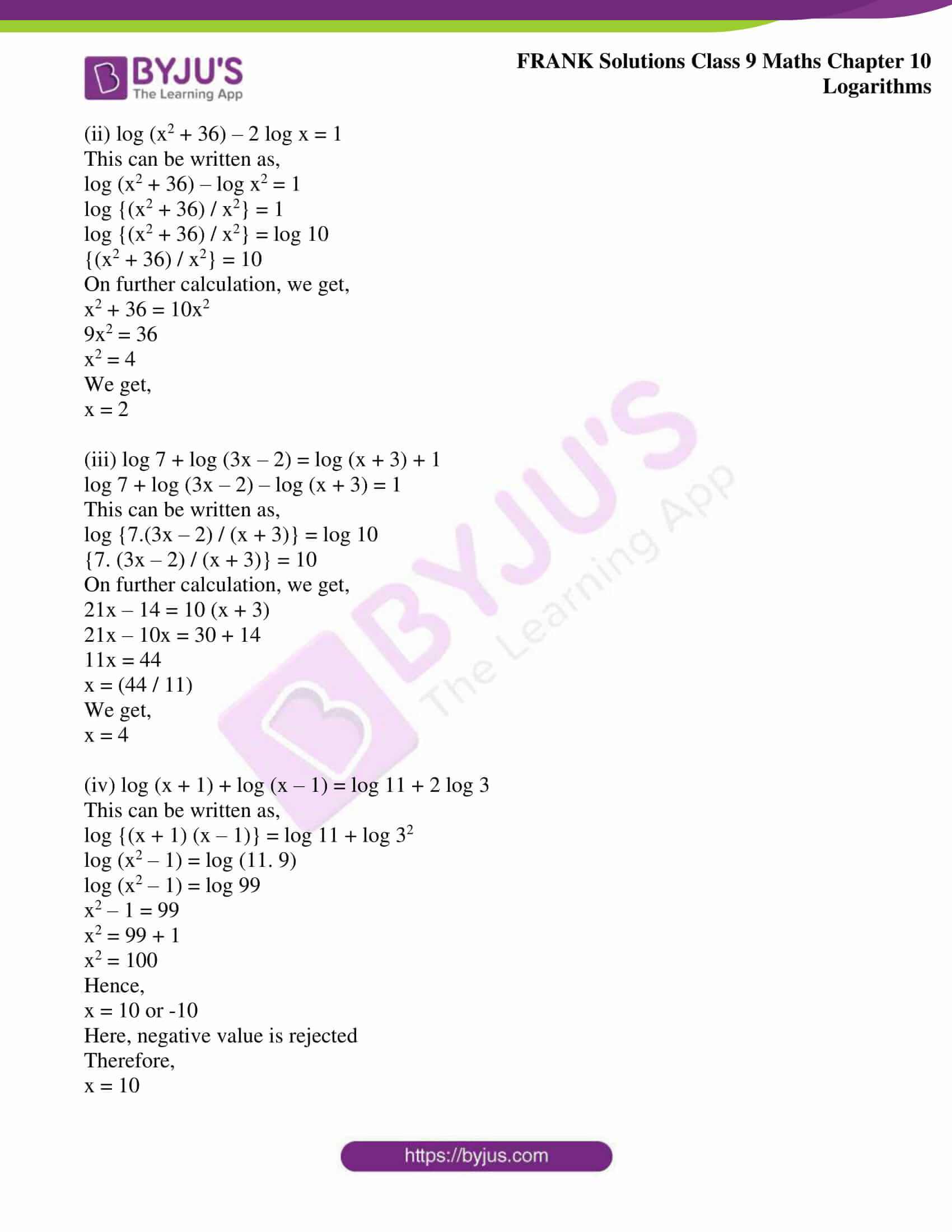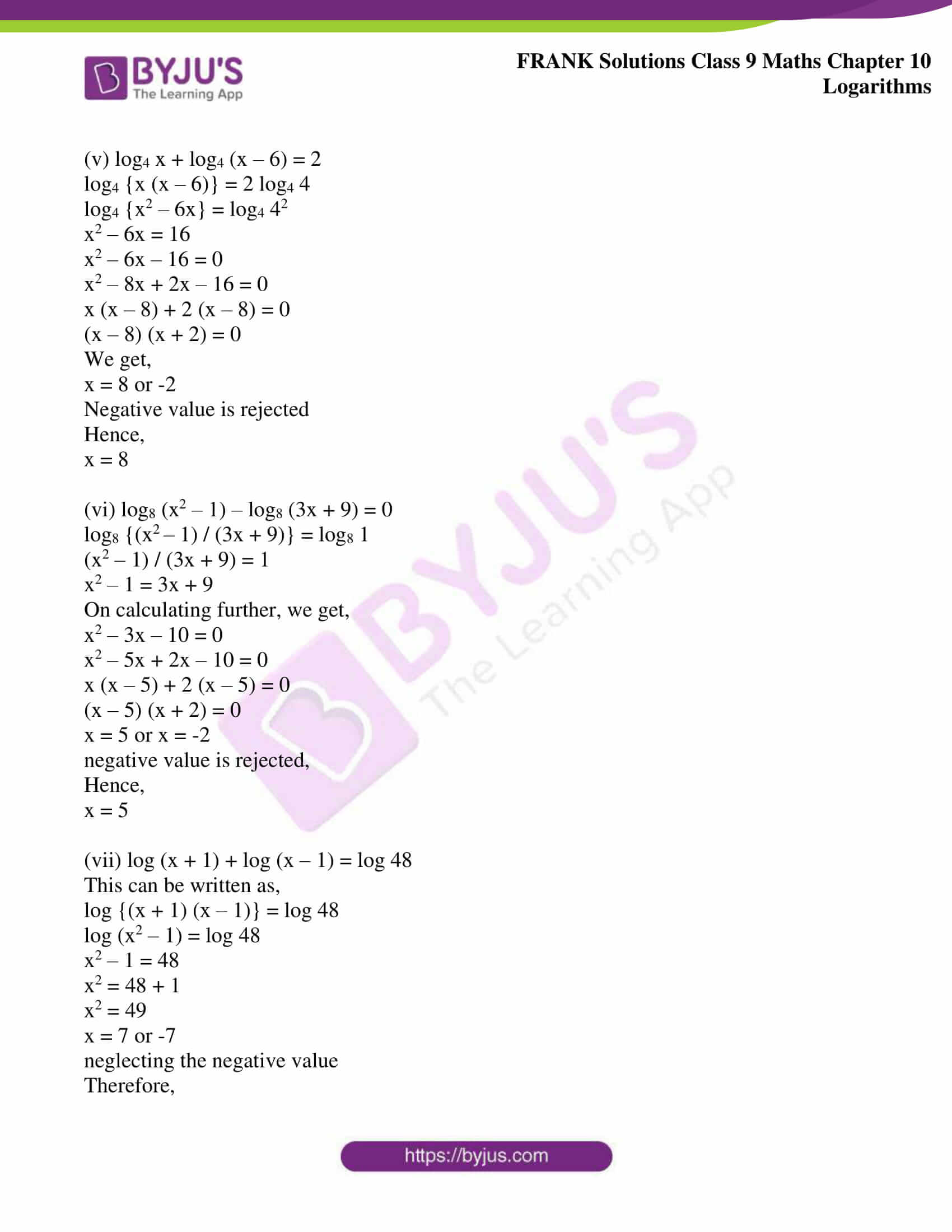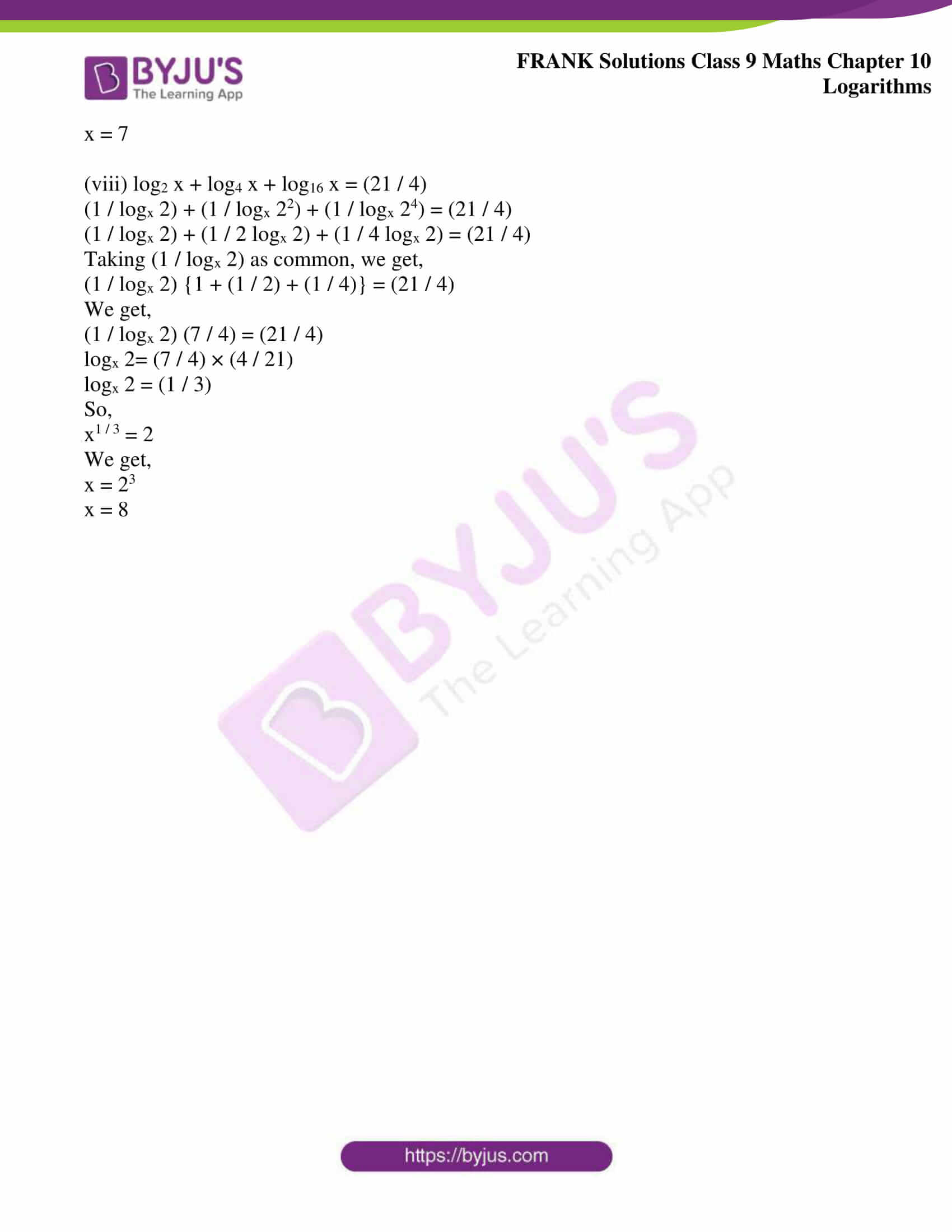## Access Frank Solutions for Class 9 Maths Chapter 10 Logarithms

1. Express each of the following in the logarithmic form:

(i) 33 = 27

(ii) 54 = 625

(iii) 90 = 1

(iv) (1 / 8) = 2-3

(v) 112 = 121

(vi) 3-2 = (1 / 9)

(vii) 10-4 = 0.0001

(viii) 70 = 1

(ix) (1 / 3)4 = (1 / 81)

(x) 9– 4 = (1 / 6561)

Solution:

The logarithmic forms of the given expressions are as follows:

(i) 33 = 27

log3 27 = 3

(ii) 54 = 625

log5 625 = 4

(iii) 90 = 1

log9 1 = 0

(iv) (1 / 8) = 2– 3

log2 (1 / 8) = – 3

(v) 112 = 121

log11 121 = 2

(vi) 3-2 = (1 / 9)

log3 (1 / 9) = – 2

(vii) 10-4 = 0.0001

log10 0.0001 = – 4

(viii) 70 = 1

log7 1 = 0

(ix) (1 / 3)4 = (1 / 81)

log1 / 3 (1 / 81) = 4

(x) 9-4 = (1 / 6561)

log9 (1 / 6561) = – 4

2. Express each of the following in the exponential form:

(i) log2 128 = 7

(ii) log3 81 = 4

(iii) log10 0.001 = – 3

(iv) log2 (1 / 32) = – 5

(v) logb a = c

(vi) log2 (1 / 2) = – 1

(vii) log5 a = 3

(viii)(ix)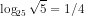(x)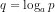(xi)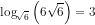(xii) – 2 = log2 (0.25)

Solution:

(i) log2 128 = 7

128 = 27

Hence, the exponential form of log2 128 = 7 is 27

(ii) log3 81 = 4

81 = 34

Hence, the exponential form of log3 81 = 4 is 34

(iii) log10 0.001 = – 3

0.001 = 10-3

Hence, the exponential form of log10 0.001 = – 3 is 10-3

(iv) log2 (1 / 32) = – 5

(1 / 32) = 2– 5

Hence, the exponential form of log2 (1 / 32) = – 5 is 2-5

(v) logb a = c

a = bc

Hence, the exponential form of logb a = c is bc

(vi) log2 (1 / 2) = – 1

(1 / 2) = 2– 1

Hence, the exponential form of log2 (1 / 2) = – 1 is 2-1

(vii) log5 a = 3

a = 53

Hence, the exponential form of log5 a = 3 is 53

(viii)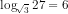27 =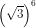Hence, the exponential form ofis(ix)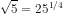Hence, the exponential form ofis 251 / 4

(x)p = aq

Hence, the exponential form ofis aq

(xi)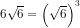Hence, the exponential form ofis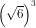(xii) -2 =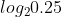We get,

2-2 = 0.25

3. Find x in each of the following when:

(i) logx 49 = 2

(ii) logx 125 = 3

(iii) logx 243 = 5

(iv) log8 x = (2 / 3)

(v) log7 x = 3

(vi) log4 x = – 4

(vii) log2 0.5 = x

(viii) log3 243 = x

(ix) log10 0.0001 = x

(x) log4 0.0625 = x

Solution:

(i) logx 49 = 2

x2 = 49

x = 7

Therefore, the value of x is 7

(ii) logx 125 = 3

x3 = 125

x3 = 53

x = 5

Therefore, the value of x is 5

(iii) logx 243 = 5

x5 = 243

x5 = 35

x = 3

Therefore, the value of x is 3

(iv) log8 x = (2 / 3)

x = 82 / 3

Taking the cube on both sides, we get,

x3 = 82

x3 = 64

x3 = 43

x = 4

Therefore, the value of x is 4

(v) log7 x = 3

x = 73

x = 343

Therefore, the value of x is 343

(vi) log4 x = – 4

x = 4– 4

x = (1 / 256)

Therefore, the value of x is (1 / 256)

(vii) log2 0.5 = x

2x = 0.5

2x = (1 / 2)

2x = 2-1

x = – 1

Therefore, the value of x is -1

(viii) log3 243 = x

243 = 3x

35 = 3x

x = 5

Therefore, the value of x is 5

(ix) log10 0.0001 = x

0.0001 = 10x

10x = 10– 4

x = – 4

Therefore, the value of x is -4

(x) log4 0.0625 = x

0.0625 = 4x

4x = 4– 2

x = – 2

Therefore, the value of x is -2

4. Find the values of:

(i) log10 1000

(ii) log3 81

(iii) log5 3125

(iv) log2 128

(v) log1 / 5 125

(vi) log10 0.0001

(vii) log5 125

(viii) log8 2

(ix) log1 / 2 16

(x) log0.01 10

(xi) log3 81

(xii) log5 (1 / 25)

(xiii) log2 8

(xiv) loga a3

(xv) log0.1 10

(xvi)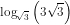Solution:

(i) log10 1000

Let log10 1000 = x

10x = 1000

10x = 103

We get,

x = 3

Hence, the value of x is 3

(ii) log3 81

Let log3 81 = x

3x = 81

3x = 34

We get,

x = 4

Hence, the value of x is 4

(iii) log5 3125

Let log5 3125 = x

5x = 3125

5x = 55

We get,

x = 5

Hence, the value of x is 5

(iv) log2 128

Let log2 128 = x

2x = 128

2x = 27

We get,

x = 7

Hence, the value of x is 7

(v) log1 / 5 125

Let log1 / 5 125 = x

(1 / 5)x = 125

5– x = 53

– x = 3

We get,

x = – 3

Hence, the value of x is -3

(vi) log10 0.0001

Let log10 0.0001 = x

0.0001 = 10x

10x = 10– 4

We get,

x = – 4

Hence, the value of x is -4

(vii) log5 125

Let log5 125 = x

125 = 5x

5x = 53

We get,

x = 3

Hence, the value of x is 3

(viii) log8 2

Let log8 2 = x

2 = 8x

This can be written as,

(23)x = 2

23x = 21

3x = 1

We get,

x = (1 / 3)

Hence, the value of x is (1 / 3)

(ix) log1 / 2 16

Let log1 / 2 16 = x

16 = (1 / 2)x

2– x = 24

– x = 4

We get,

x = -4

Hence, the value of x is -4

(x) log0.01 10

Let log0.01 10 = x

(0.01)x = 10

(10-2)x = 101

10-2x = 101

-2x = 1

We get,

x = (- 1 / 2)

Hence, the value of x is (-1 / 2)

(xi) log3 81

Let log3 81 = x

3x = 81

3x = 34

We get,

x = 4

Hence, the value of x is 4

(xii) log5 (1 / 25)

Let log5 (1 / 25) = x

5x = (1 / 25)

5x = 5-2

We get,

x = -2

Hence, the value of x is -2

(xiii) log2 8

Let log2 8 = x

2x = 8

2x = 23

We get,

x = 3

Hence, the value of x is 3

(xiv) loga a3

Let loga a3 = x

ax = a3

We get,

x = 3

Hence, the value of x is 3

(xv) log0.1 10

Let log0.1 10 = x

(0.1)x = 10

(10-1)x = 101

-x = 1

We get,

x = -1

Hence, the value of x is -1

(xvi)Let= x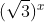= 33

3x / 2 = 31 + 1 / 2

3x / 2 = 33 / 2

(x / 2) = (3 / 2)

We get,

x = 3

Hence, the value of x is 3

5. If log10 x = a, express the following in terms of x:

(i) 102a

(ii) 10a + 3

(iii) 10– a

(iv) 102a – 3

Solution:

(i) 102a

log10 x = a

x = 10a

Hence,

102a = (10a)2

102a = x2

(ii) 10a + 3

log10 x = a

x = 10a

Hence,

10a + 3 = 10a. 103

10a + 3 = x.1000

10a + 3 = 1000x

(iii) 10-a

log10 x = a

x = 10a

Hence,

10-a = x-1

10-a = (1 / x)

(iv) 102a – 3

log10 x = a

x = 10a

Hence,

102a – 3 = 102a.10-3

102a – 3 = (10a)2 10– 3

102a – 3 = (x2 / 1000)

6. If log10 m = n, express the following in terms of m:

(i) 10n – 1

(ii) 102n – 1

(iii) 10– 3n

Solution:

(i) 10n – 1

log10 m = n

m = 10n

Therefore,

10n – 1 = 10n.10– 1

10n – 1 = (m / 10) [m = 10n]

(ii) 102n + 1

log10 m = n

m = 10n

Therefore,

102n + 1 = 102n. 101

102n + 1 = (10n)2 . 10

102n + 1 = (m)2 . 10 [m = 10n]

102n + 1 = 10m2

(iii) 10-3n

log10 m = n

m = 10n

Therefore,

10-3n = (10n)– 3

10-3n = (m)-3 [m = 10n]

10-3n = (1 / m3)

7. If log10 x = p, express the following in terms of x:

(i) 10p

(ii) 10p + 1

(iii) 102p – 3

(iv) 102 – p

Solution:

(i) 10p

log10 x = p

We get,

x = 10p

(ii) 10p + 1

log10 x = p

x = 10p

Therefore,

10p + 1 = 10p.101

10p + 1 = (x). 10 [x = 10p]

We get,

10p + 1 = 10x

(iii) 102p – 3

log10 x = p

x = 10p

Therefore,

102p – 3 = 102p. 10-3

102p – 3 = (10p)2. 10-3

102p – 3 = (x)2.10-3 [x = 10p]

102p – 3 = (x2 / 1000)

(iv) 102 – p

log10 x = p

x = 10p

Therefore,

102 – p = 102.10-p

102 – p = 100.x-1

102 – p = (100 / x)

8. If log10 x = a, log10 y = b and log10 z = 2a – 3b, express z in terms of x and y.

Solution:

log10 x = a

x = 10a

log10 y = b

y = 10b

log10 z = 2a – 3b

z = 102a – 3b

Therefore,

z = 102a – 3b

z = (10a)2.(10b)-3

z = (x)2.(y)-3

z = (x2 / y3)

9. Express the following in terms of log 2 and log 3:

(i) log 36

(ii) log 54

(iii) log 144

(iv) log 216

(v) log 648

(vi) log 128

Solution:

(i) log 36

log 36 = log (2 × 2 × 3 × 3)

log 36 = log (22 × 32)

log 36 = log 22 + log 32

We get,

log 36 = 2 log 2 + 2 log 3

(ii) log 54

log 54 = log (2 × 3 × 3 × 3)

log 54 = log (2 × 33)

log 54 = log 2 + log 33

We get,

log 54 = log 2 + 3 log 3

(iii) log 144

log 144 = log (24 × 32)

log 144 = log 24 + log 32

We get,

log 144 = 4 log 2 + 2 log 3

(iv) log 216

log 216 = log (23 × 33)

log 216 = log 23 + log 33

We get,

log 216 = 3 log 2 + 3 log 3

(v) log 648

log 648 = log (23 × 34)

log 648 = log 23 + log 34

We get,

log 648 = 3 log 2 + 4 log 3

(vi) log 128

log 128 = log (3 × 22)8

log 128 = 8 log (3 × 22)

log 128 = 8 {log 3 + log 22}

We get,

log 128 = 8 {log 3 + 2 log 2}

10. Express the following in terms of log 5 and/or log 2:

(i) log 20

(ii) log 80

(iii) log 125

(iv) log 160

(v) log 500

(vi) log 250

Solution:

(i) log 20

log 20 = log (22 × 5)

log 20 = log 22 + log 5

We get,

log 20 = 2 log 2 + log 5

(ii) log 80

log 80 = log (24 × 5)

log 80 = log 24 + log 5

We get,

log 80 = 4 log 2 + log 5

(iii) log 125

log 125 = log 53

We get,

log 125 = 3 log 5

(iv) log 160

log 160 = log (25 × 5)

log 160 = log 25 + log 5

We get,

log 160 = 5 log 2 + log 5

(v) log 500

log 500 = log (22 × 53)

log 500 = log 22 + log 53

We get,

log 500 = 2 log 2 + 3 log 5

(vi) log 250 = log (53 × 2)

log 250 = log 53 + log 2

We get,

log 250 = 3 log 5 + log 2

11. Express the following in terms of log 2 and log 3:

(i)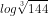(ii)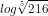(iii)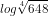(iv) log (26 / 51) – log (91 / 119)

(v) log (225 / 16) – 2 log (5 / 9) + log (2 / 3)5

Solution:

(i)= log (144)1 / 3

= (1 / 3) log 144

= (1 / 3) log (24 × 32)

= (1 / 3) log 24 + (1 / 3) log 32

We get,

= (4 / 3) log 2 + (2 / 3) log 3

(ii)= log (216)1 / 5

= (1 / 5) log 216

= (1 / 5) log (23 × 33)

= (1 / 5) log 23 + (1 / 5) log 33

We get,

= (3 / 5) log 2 + (3 / 5) log 3

(iii)= log (648)1 / 4

= (1 / 4) log 648

= (1 / 4) log (23 × 34)

= (1 / 4) log 23 + (1 / 4) log 34

= (3 / 4) log 2 + (4 / 4) log 3

We get,

= (3 / 4) log 2 + 1 log 3

= (3 / 4) log 2 + log 3

(iv) log (26 / 51) – log (91 / 119)

= log {(2 × 13) / (3 × 17)} – log {(7 x 13) / (7 x 17)}

= log {(2 × 13) / (3 × 17)} – log (13 / 17)

= (log 13 + log 2 – log 3 – log 17) – (log 13 – log 17)

= log 13 + log 2 – log 3 – log 17 – log 13 + log 17

We get,

= log 2 – log 3

(v) log (225 / 16) – 2 log (5 / 9) + log (2 / 3)5

= log (225 / 16) – 2 log (5 / 9) + 5 log (2 / 3)

= log 225 – log 16 – 2 {log 5 – log 9} + 5 {log 2 – log 3}

= log (52 × 32) – log 24 – 2 {log 5 – log 32} + 5 {log 2 – log 3}

= log 52 + log 32 – 4 log 2 – 2 {log 5 – 2 log 3} + 5 {log 2 – log 3}

= 2 log 5 + 2 log 3 – 4 log 2 – 2 log 5 + 4 log 3 + 5 log 2 – 5 log 3

We get,

= log 2 + log 3

12. Write the logarithmic equation for:

(i) F = {G (m1m2) / d2}

(ii) E = (1 / 2) mv2

(iii)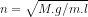(iv) V = (4 / 3) πr3

(v)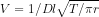Solution:

(i) F = {G (m1m2) / d2}

Taking log on both sides, we get,

log F = log [{G (m1m2)} / d2]

log F = log (Gm1m2) – log d2

We get,

log F = log G + log m1 + log m2 – 2 log d

(ii) E = (1 / 2) mv2

Taking log on both sides, we get,

log E = log {(1 / 2) mv2}

log E = log (1 / 2) + log m + log v2

We get,

log E = log 1 – log 2 + log m + 2 log v

(iii)n = (M.g / m.l)1 / 2

On taking log on both sides, we get,

log n = log (M.g / m.l)1 / 2

log n = (1 / 2) log (M.g / m. l)

log n = (1 / 2) {log (M.g) – log (m.l)}

We get,

log n = (1 / 2) {log M + log g – log m – log l}

(iv) V = (4 / 3) πr3

On taking log on both sides, we get,

log V = log {(4 / 3)πr3}

log V = log 4 + log π + log r3 – log 3

log V = log 22 + log π + 3 log r – log 3

log V = 2 log 2 – log 3 + log π + 3 log r

(v)V = 1 / Dl (T / πr)1 / 2

On taking log on both sides, we get,

log V = log {1 / Dl (T / πr)1 / 2}

log V = log (1 / Dl) + log (T / πr)1 / 2

log V = (log 1 – log D – log l) + (1 / 2) log (T / πr)

log V = (0 – log D – log l) + (1 / 2) {(log T – log π – log r)}

We get,

log V = (1 / 2) (log T – log π – log r) – log D – log l

13. Express the following as a single logarithm:

(i) log 18 + log 25 – log 30

(ii) log 144 – log 72 + log 150 – log 50

(iii) 2 log 3 – (1 / 2) log 16 + log 12

(iv) 2 + (1 / 2) log 9 – 2 log 5

(v) 2 log (9 / 5) – 3 log (3 / 5) + log (16 / 20)

(vi) 2 log (15 / 18) – log (25 / 162) + log (4 / 9)

(vii) 2 log (16 / 25) – 3 log (8 / 5) + log 90

(viii) (1 / 2) log 25 – 2 log 3 + log 36

(ix) log (81 / 8) – 2 log (3 / 5) + 3 log (2 / 5) + log (25 / 9)

(x) 3 log (5 / 8) + 2 log (8 / 15) – (1 / 2) log (25 / 81) + 3

Solution:

(i) log 18 + log 25 – log 30

This can be written as,

= log (2 × 32) + log 52 – log (2 × 3 × 5)

= log 2 + log 32 + 2 log 5 – {log 2 + log 3 + log 5}

= log 2 + 2 log 3 + 2 log 5 – log 2 – log 3 – log 5

= log 3+ log 5

= log (3 × 5)

We get,

= log 15

(ii) log 144 – log 72 + log 150 – log 50

This can be written as,

= log (24 × 32) – log (23 × 32) + log (2 × 3 × 52) – log (2 × 52)

= log 24 + log 32 – {log 23 + log 32) + log 2 + log 3 + log 52 – {log 2 + log 52}

= 4 log 2 + 2 log 3 – 3 log 2 – 2 log 3 + log 2 + log 3 + 2 log 5 – log 2 – 2 log 5

We get,

= log 2 + log 3

= log (2 × 3)

We get,

= log 6

(iii) 2 log 3 – (1 / 2) log 16 + log 12

= 2 log 3 – (1 / 2) log 24 + log (22 × 3)

= 2 log 3 – (1 / 2) × 4 log 2 + log 22 + log 3

We get,

= 2 log 3 – 2 log 2 + 2 log 2 + log 3

= 3 log 3

= log 33

We get,

= log 27

(iv) 2 + (1 / 2) log 9 – 2 log 5

This can be written as,

= 2 + (1 / 2) log 32 – 2 log 5

= 2 log 10 + (1 / 2) × 2 log 3 – 2 log 5

= log 102 + log 3 – log 52

= log 100 + log 3 – log 25

= log {(100 × 3) / 25}

We get,

= log 12

(v) 2 log (9 / 5) – 3 log (3 / 5) + log (16 / 20)

= 2 log 9 – 2 log 5 – 3 log 3 + 3 log 5 + log 16 – log 20

This can be written as,

= 2 log (32) – 2 log 5 – 3 log 3 + 3 log 5 + log (42) – log (5 × 4)

= 4 log 3 – 2 log 5 – 3 log 3 + 3 log 5 + 2 log 4 – log 5 – log 4

= (4 – 3) log 3 + (-2 -1 + 3) log 5 + log 4

= log 3 + log 4

= log (3 × 4)

We get,

= log 12

(vi) 2 log (15 / 18) – log (25 / 162) + log (4 / 9)

= 2 log {5 / (2 × 3)} – log {52 / (2 × 34)} + log (22 / 32)

= 2 log 5 – 2 log 2 – 2 log 3 – {log 52 – log 2 – log 34} + log 22 – log 32

= 2 log 5 – 2 log 2 – 2 log 3 – 2 log 5 + log 2 + 4 log 3 + 2 log 2 – 2 log 3

We get,

= log 2

(vii) 2 log (16 / 25) – 3 log (8 / 5) + log 90

This can be written as,

= 2 log (24 / 52) – 3 log (23 / 5) + log (2 × 5 × 32)

= 2 log 24 – 2 log 52 – 3 {log 23 – log 5) + log 2 + log 5 + log 32

= 4 × 2 log 2 – 2 × 2 log 5 – 3 × 3 log 2 + 3 log 5 + log 2 + log 5 + 2 log 3

= 8 log 2 – 4 log 5 – 9 log 2 + 3 log 5 + log 2 + log 5 + 2 log 3

= 2 log 3

= log 32

We get,

= log 9

(viii) (1 / 2) log 25 – 2 log 3 + log 36

= (1 / 2) log 52 – 2 log 3 + log (22 × 32)

= (1 / 2) × 2 log 5 – 2 log 3 + log 22 + log 32

= log 5 – log 32 + 2 log 2 + log 32

= log 5 + 2 log 2

= log 5 + log 22

= log 5 + log 4

= log (5 × 4)

We get,

= log 20

(ix) log (81 / 8) – 2 log (3 / 5) + 3 log (2 / 5) + log (25 / 9)

= log (34 / 23) – 2 log (3 / 5) + 3 log (2 / 5) + log (52 / 32)

= log 34 – log 23 – 2 log 3 + 2 log 5 + 3 log 2 – 3 log 5 + log 52 – log 32

= 4 log 3 – 3 log 2 – 2 log 3 + 2 log 5 + 3 log 2 – 3 log 5 + 2 log 5 – 2 log 3

We get,

= log 5

(x) 3 log (5 / 8) + 2 log (8 / 15) – (1 / 2) log (25 / 81) + 3

This can be written as,

= 3 log (5 / 23) + 2 log {23 / (3 × 5)} – (1 / 2) log (52 / 34) + 3 log 10

= 3 log 5 – 3 log 23 + 2 log 23 – 2 log 3 – 2 log 5 – (1 / 2) log 52 + (1 / 2) log 34 + 3 log (2 × 5)

= 3 log 5 – 3 × 3 log 2 + 2 × 3 log 2 – 2 log 3 – 2 log 5 – (1 / 2) × 2 log 5 + (1 / 2) × 4 log 3 + 3 log 2 + 3 log 5

= 3 log 5 – 9 log 2 + 6 log 2 – 2 log 3 – 2 log 5 – log 5 + 2 log 3 + 3 log 2 + 3 log 5

= 3 log 5

= log 53

We get,

= log 125

14. Simplify the following:

(i) 2 log 5 + log 8 – (1 / 2) log 4

(ii) 2 log 7 + 3 log 5 – log (49 / 8)

(iii) 3 log (32/27) + 5 log (125/ 24) – 3 log (625/ 243) + log (2 / 75)

(iv) 12 log (3/2) + 7 log (125 / 27) – 5 log (25 / 36) – 7 log 25 + log (16 / 3)

Solution:

(i) 2 log 5 + log 8 – (1 / 2) log 4

= 2 log 5 + log 23 – (1 / 2) log 22

= 2 log 5 + 3 log 2 – (1 / 2) × 2 log 2

= 2 log 5 + 3 log 2 – log 2

= 2 log 5 + 2 log 2

= 2 (log 5 + log 2)

= 2 log (5 × 2)

We get,

= 2 log 10

= 2 × 1

= 2

(ii) 2 log 7 + 3 log 5 – log (49 / 8)

= 2 log 7 + 3 log 5 – log 49 + log 8

= 2 log 7 + 3 log 5 – log 72 + log 23

= 2 log 7 + 3 log 5 – 2 log 7 + 3 log 2

= 3 log 5 + 3 log 2

= 3 (log 5 + log 2)

= 3 log (5 × 2)

= 3 log 10

We get,

= 3 × 1

= 3

(iii) 3 log (32/27) + 5 log (125/ 24) – 3 log (625/ 243) + log (2 / 75)

= 3 log (25 / 33) + 5 log {53 / (23 x 3)} – 3 log (54 / 35) + log {2 / (3 x 52)}

= 3 log 25 – 3 log 33 + 5 log 53 – 5 log 23 – 5 log 3 – 3 log 54 + 3 log 35 + log 2 – log 3 -log 52

= 15 log 2 – 9 log 3 + 15 log 5 – 15 log 2 – 5 log 3 – 12 log 5 + 15 log 3 + log 2 – log 3 – 2 log 5

= log 2 + log 5

(iv) 12 log (3/2) + 7 log (125 / 27) – 5 log (25 / 36) – 7 log 25 + log (16 / 3)

= 12 log (3/2) + 7 log (53 / 33) – 5 log {52 / (22 x 32)} – 7 log 52 + log (24 / 3)

= 12 log 3 – 12 log 2 + 7 log 53 – 7 log 33 – 5 log 52 + 5 log 22 + 5 log 32 – 7 log 52 + log 24 – log 3

= 12 log 3 – 12 log 2 + 21 log 5 – 21 log 3 – 10 log 5 + 10 log 2 + 10 log 3 – 14 log 5 + 4 log 2 – log 3

We get,

= 2log 2 – 3 log 5

15. Solve the following:

(i) log (3 – x) – log (x – 3) = 1

(ii) log (x2 + 36) – 2 log x = 1

(iii) log 7 + log (3x – 2) = log (x + 3) + 1

(iv) log (x + 1) + log (x – 1) = log 11 + 2 log 3

(v) log4 x + log4 (x – 6) = 2

(vi) log8 (x2 – 1) – log8 (3x + 9) = 0

(vii) log (x + 1) + log (x – 1) = log 48

(viii) log2 x + log4 x + log16 x = (21 / 4)

Solution:

(i) log (3 – x) – log (x – 3) = 1

This can be written as,

log {(3 – x) / (x – 3)} = 1

log {(3 – x) / (x – 3)} = log 10

(3 – x) / (x – 3) = 10

On calculating further, we get,

(3 – x) = 10 (x – 3)

(3 – x) = 10 x – 30

11x = 33

We get,

x = 3

(ii) log (x2 + 36) – 2 log x = 1

This can be written as,

log (x2 + 36) – log x2 = 1

log {(x2 + 36) / x2} = 1

log {(x2 + 36) / x2} = log 10

{(x2 + 36) / x2} = 10

On further calculation, we get,

x2 + 36 = 10x2

9x2 = 36

x2 = 4

We get,

x = 2

(iii) log 7 + log (3x – 2) = log (x + 3) + 1

log 7 + log (3x – 2) – log (x + 3) = 1

This can be written as,

log {7.(3x – 2) / (x + 3)} = log 10

{7. (3x – 2) / (x + 3)} = 10

On further calculation, we get,

21x – 14 = 10 (x + 3)

21x – 10x = 30 + 14

11x = 44

x = (44 / 11)

We get,

x = 4

(iv) log (x + 1) + log (x – 1) = log 11 + 2 log 3

This can be written as,

log {(x + 1) (x – 1)} = log 11 + log 32

log (x2 – 1) = log (11. 9)

log (x2 – 1) = log 99

x2 – 1 = 99

x2 = 99 + 1

x2 = 100

Hence,

x = 10 or -10

Here, the negative value is rejected

Therefore,

x = 10

(v) log4 x + log4 (x – 6) = 2

log4 {x (x – 6)} = 2 log4 4

log4 {x2 – 6x} = log4 42

x2 – 6x = 16

x2 – 6x – 16 = 0

x2 – 8x + 2x – 16 = 0

x (x – 8) + 2 (x – 8) = 0

(x – 8) (x + 2) = 0

We get,

x = 8 or -2

The negative value is rejected

Hence,

x = 8

(vi) log8 (x2 – 1) – log8 (3x + 9) = 0

log8 {(x2 – 1) / (3x + 9)} = log8 1

(x2 – 1) / (3x + 9) = 1

x2 – 1 = 3x + 9

On calculating further, we get,

x2 – 3x – 10 = 0

x2 – 5x + 2x – 10 = 0

x (x – 5) + 2 (x – 5) = 0

(x – 5) (x + 2) = 0

x = 5 or x = -2

The negative value is rejected,

Hence,

x = 5

(vii) log (x + 1) + log (x – 1) = log 48

This can be written as,

log {(x + 1) (x – 1)} = log 48

log (x2 – 1) = log 48

x2 – 1 = 48

x2 = 48 + 1

x2 = 49

x = 7 or -7

neglecting the negative value

Therefore,

x = 7

(viii) log2 x + log4 x + log16 x = (21 / 4)

(1 / logx 2) + (1 / logx 22) + (1 / logx 24) = (21 / 4)

(1 / logx 2) + (1 / 2 logx 2) + (1 / 4 logx 2) = (21 / 4)

Taking (1 / logx 2) as common, we get,

(1 / logx 2) {1 + (1 / 2) + (1 / 4)} = (21 / 4)

We get,

(1 / logx 2) (7 / 4) = (21 / 4)

logx 2= (7 / 4) × (4 / 21)

logx 2 = (1 / 3)

So,

x1 / 3 = 2

We get,

x = 23

x = 8